# RS Aggarwal Solutions for Class 7 Maths Exercise 3D Chapter 3 Decimals

RS Aggarwal Solutions for Class 7 Maths Exercise 3D Chapter 3 Decimals in simple PDF are given here. This exercise of RS Aggarwal Solutions for Class 7 Chapter 3 contains questions related to the Division of Decimals by 10, 100 and 1000, dividing a decimal by a whole number. On dividing a decimal by 10, 100 and 1000 the decimal point is shifted to the left by one, two and three places respectively. Students are suggested to try solving the questions from RS Aggarwal Solutions for Class 7 Maths Chapter 3 Decimals.

## Download the PDF of RS Aggarwal Solutions For Class 7 Maths Chapter 3 Decimals – Exercise 3D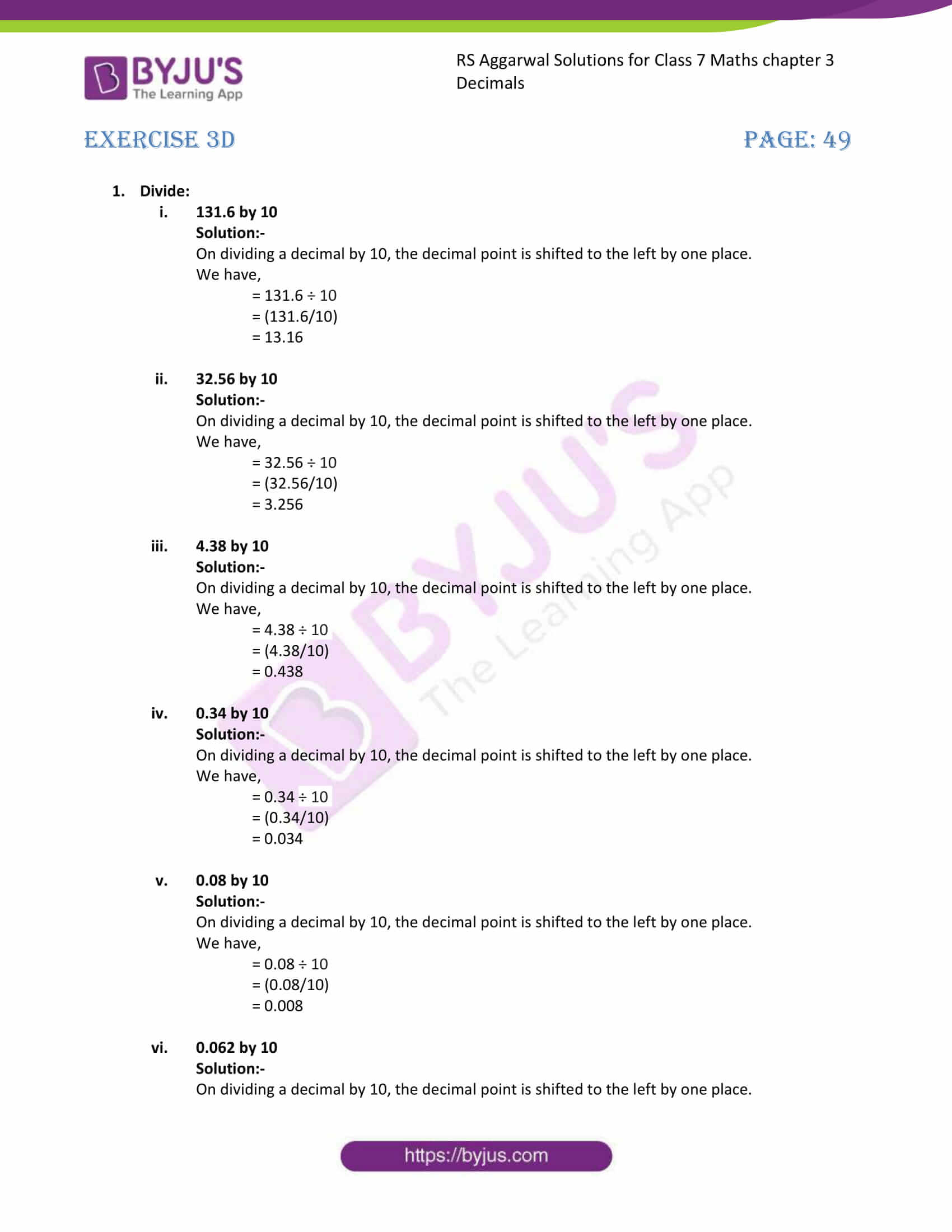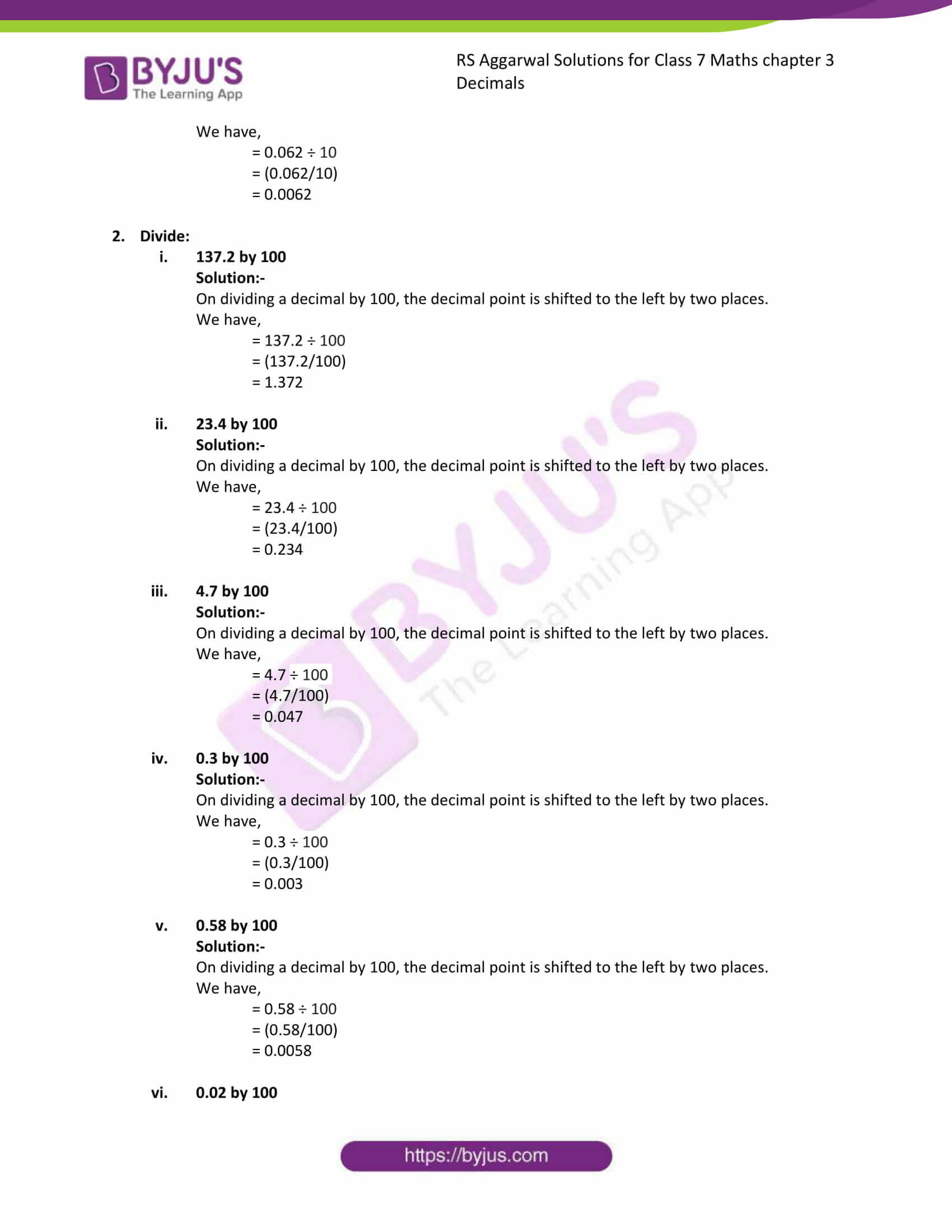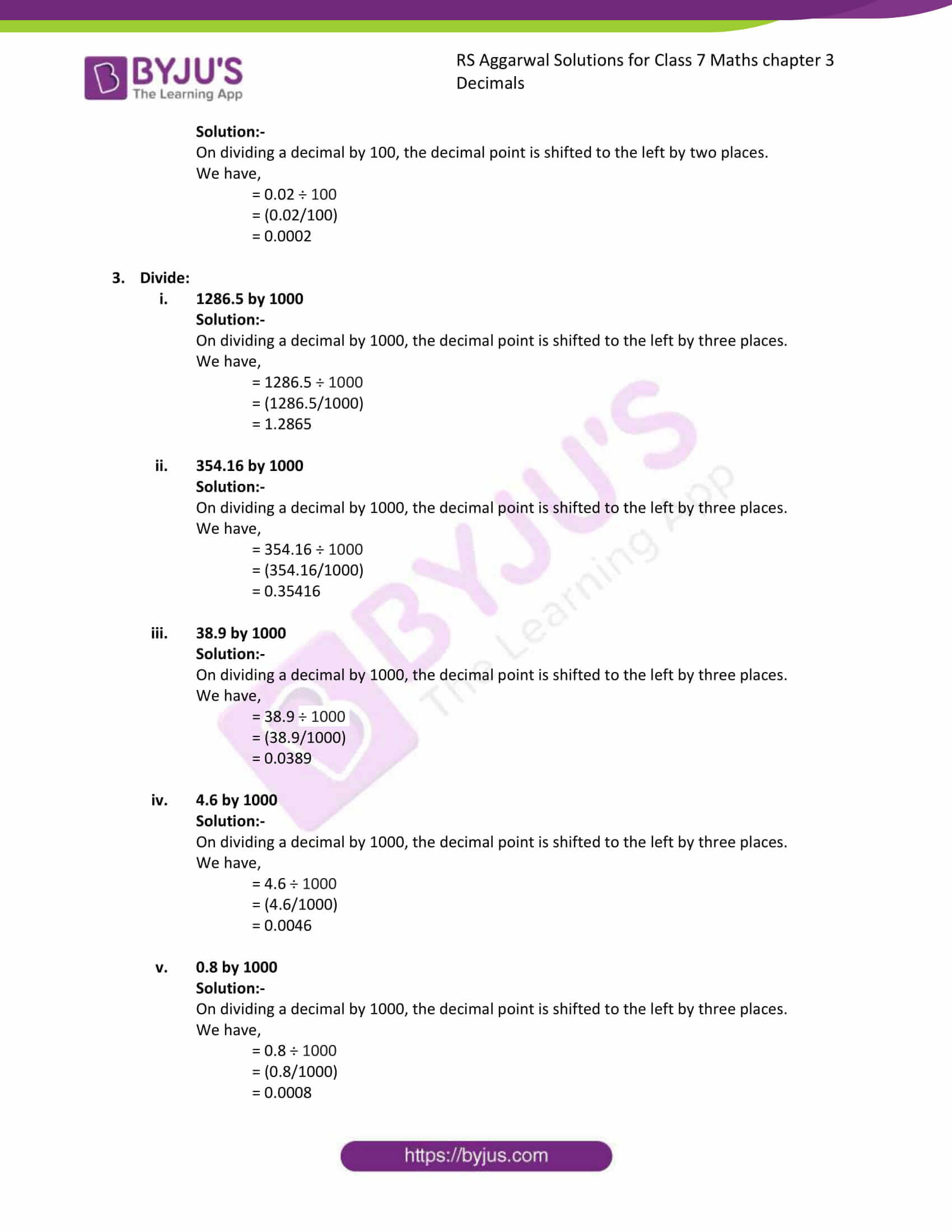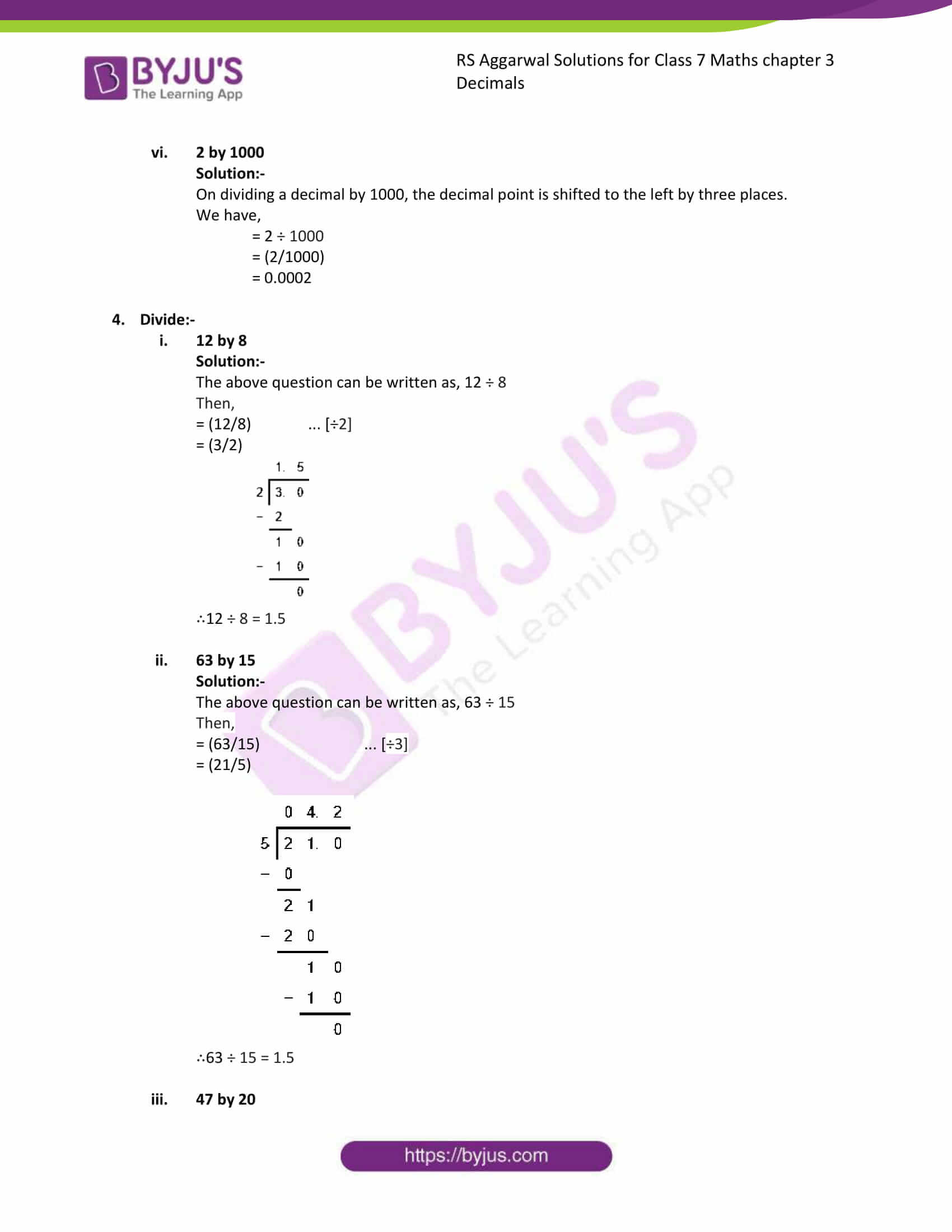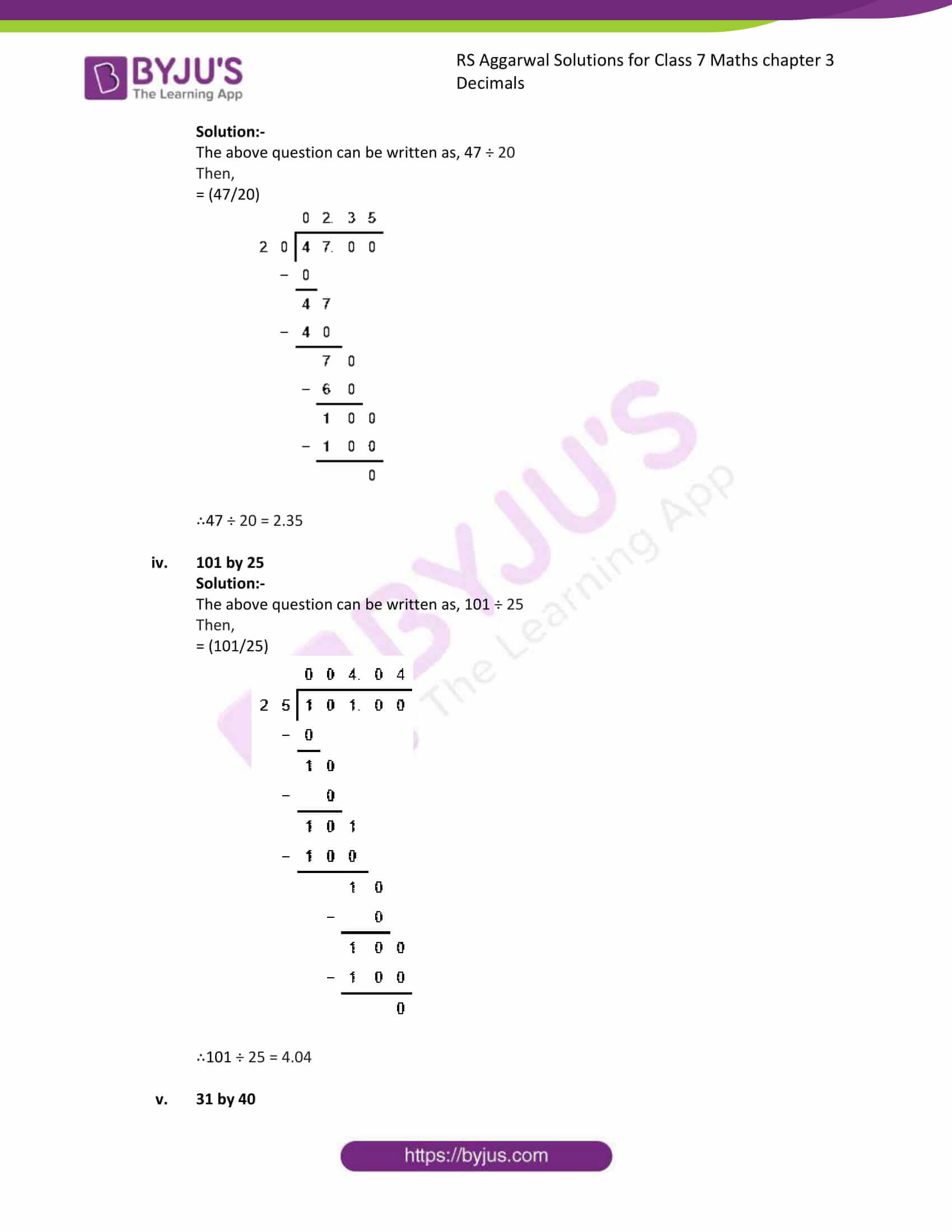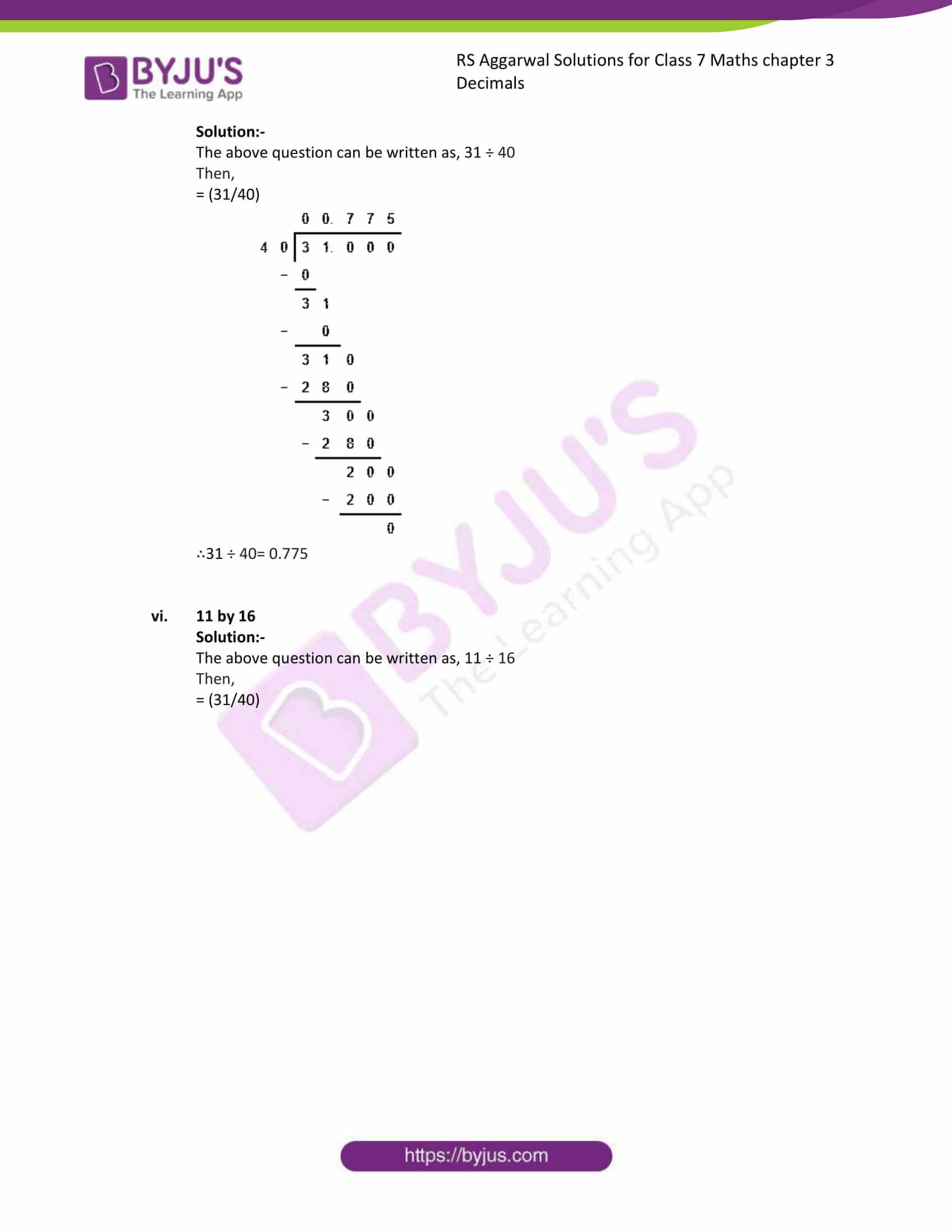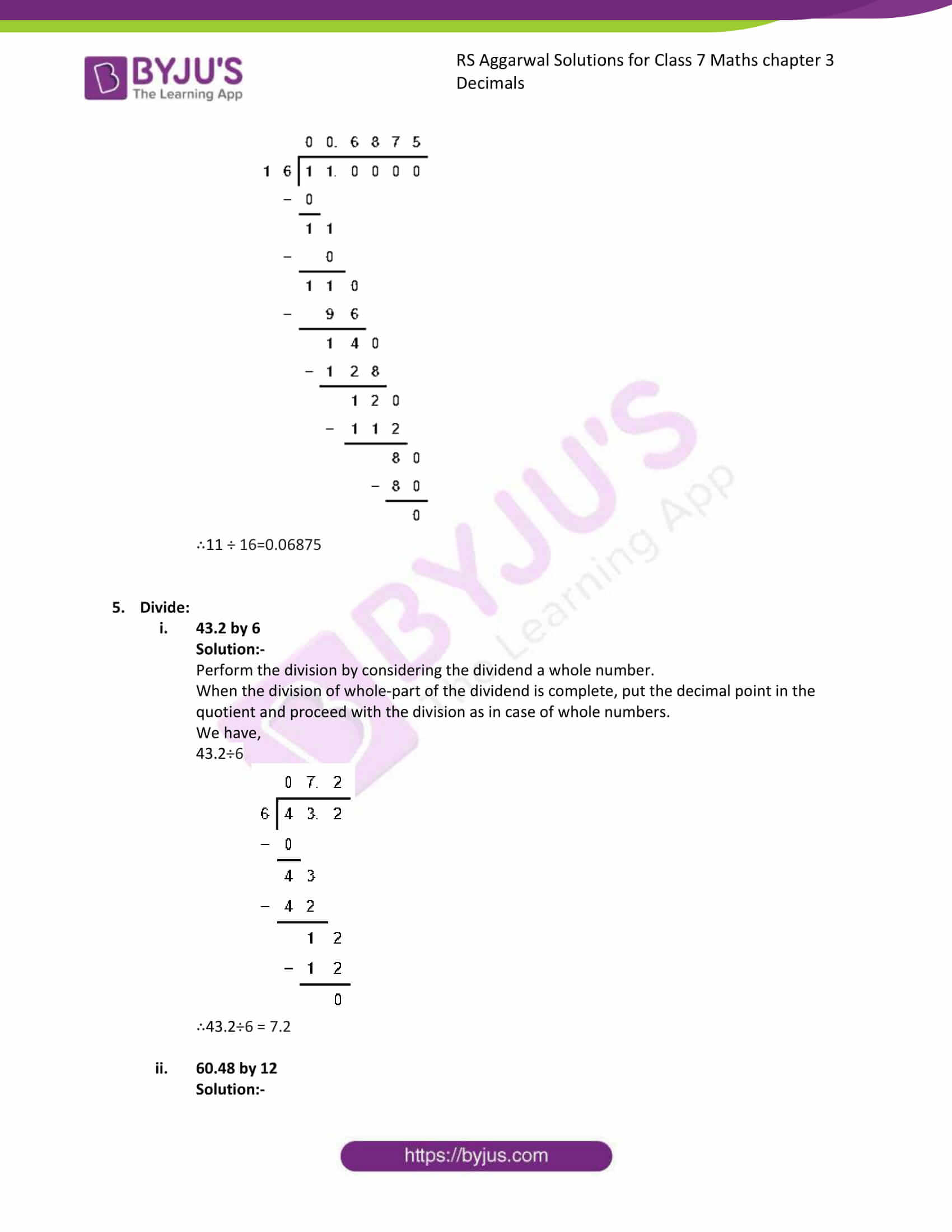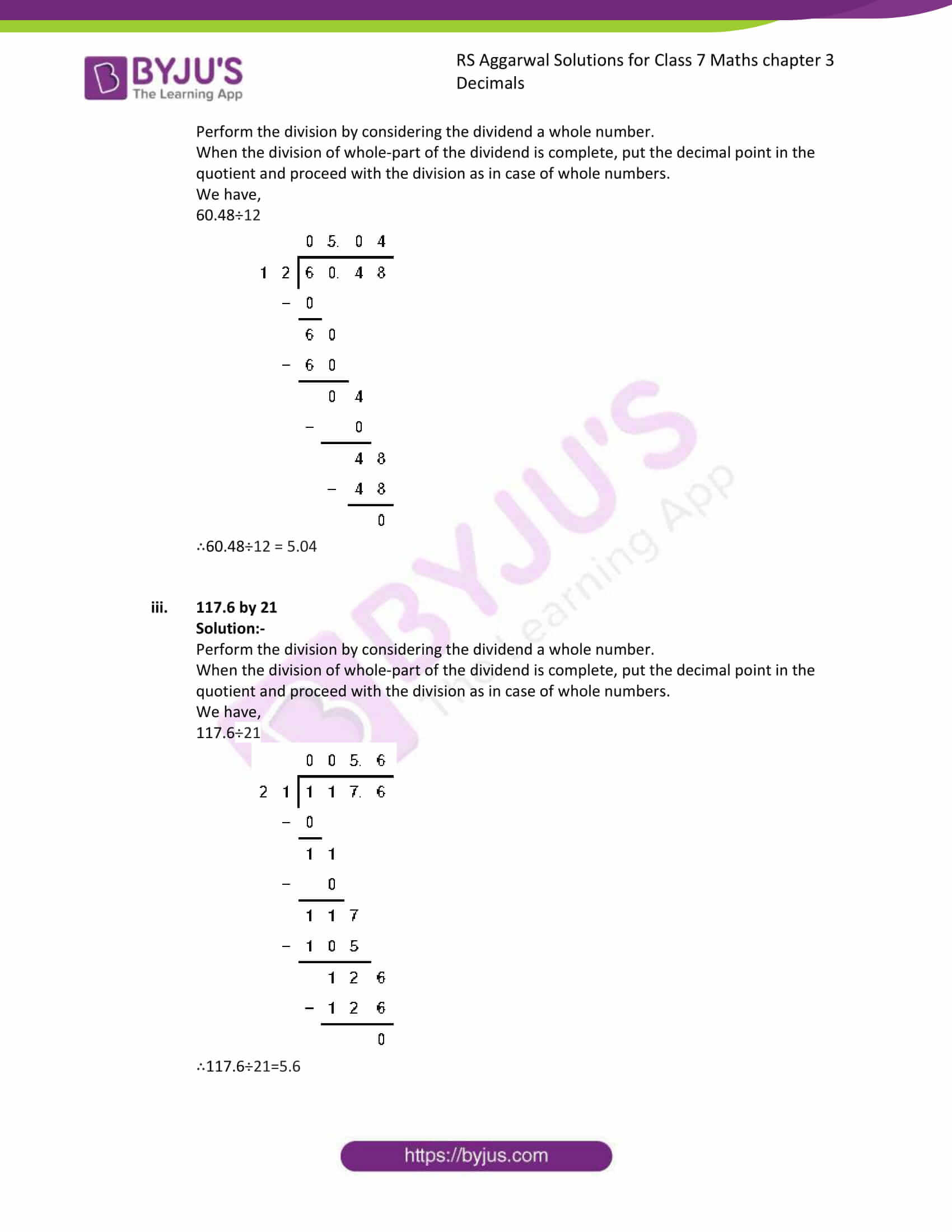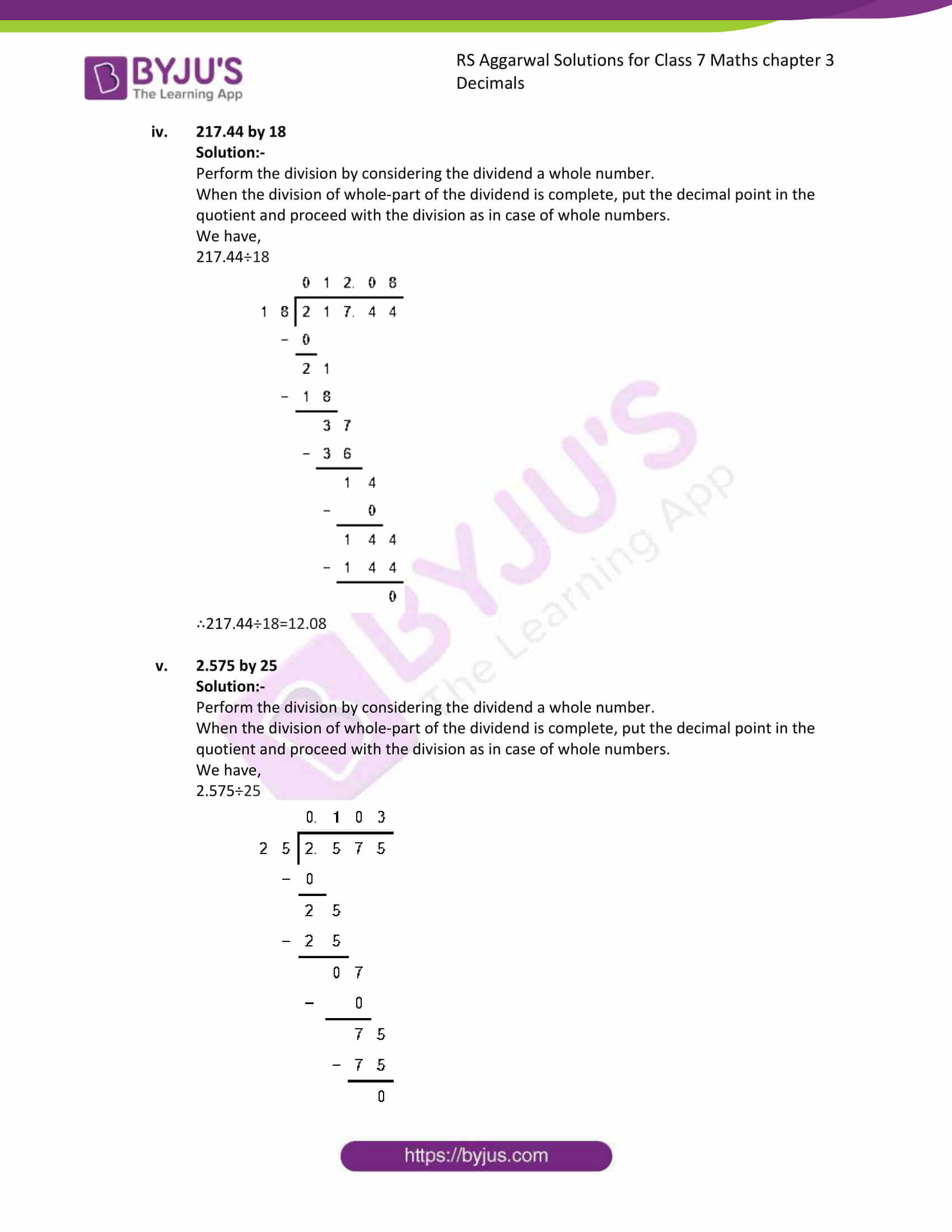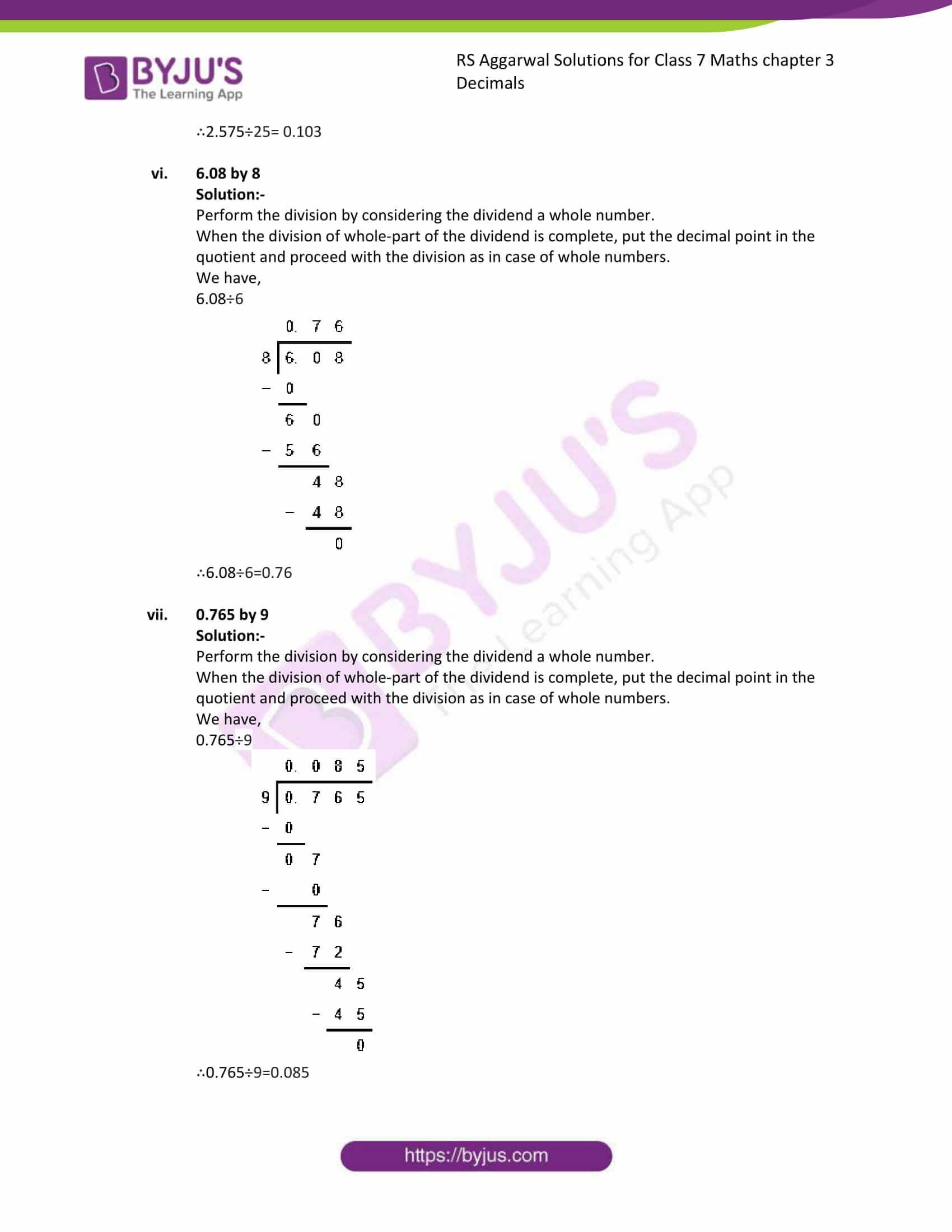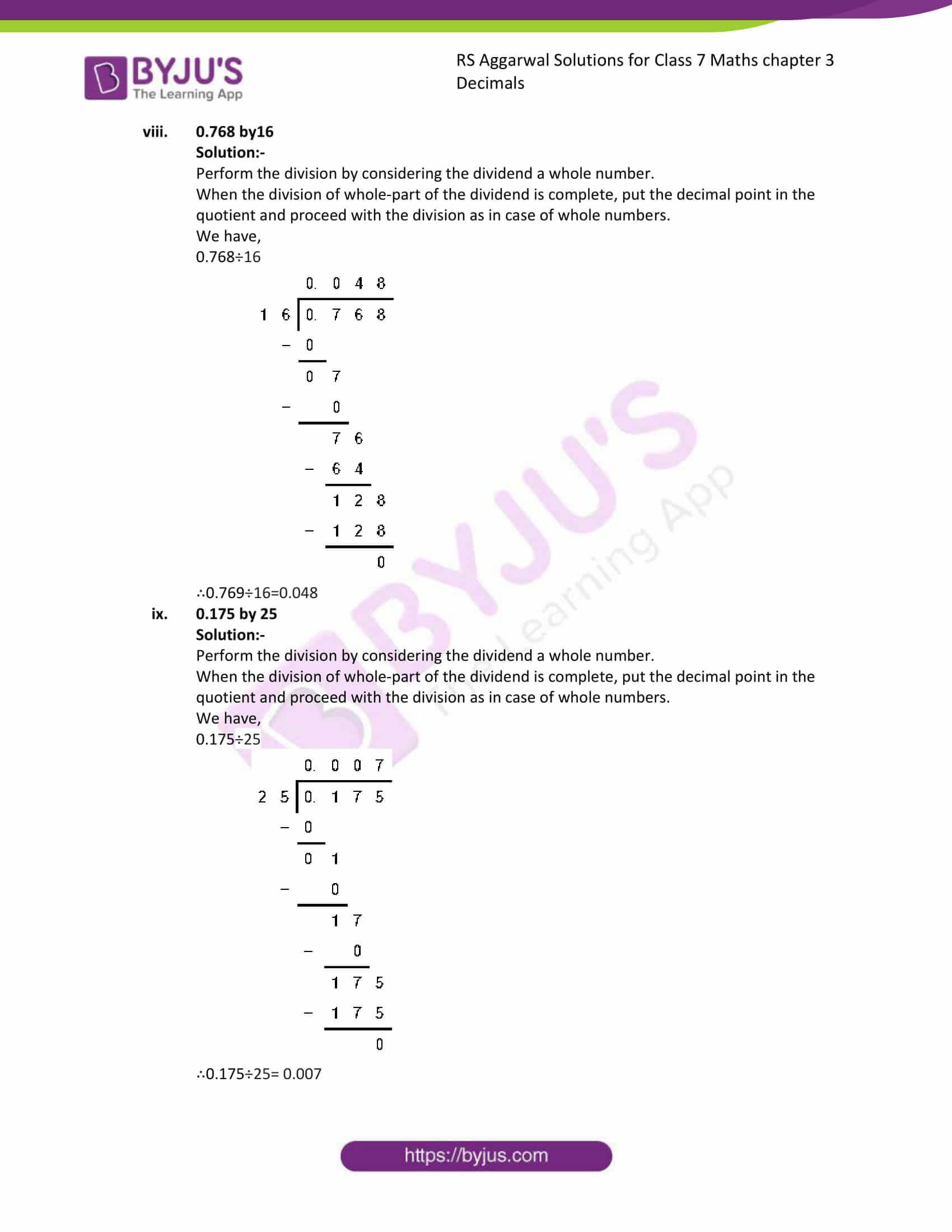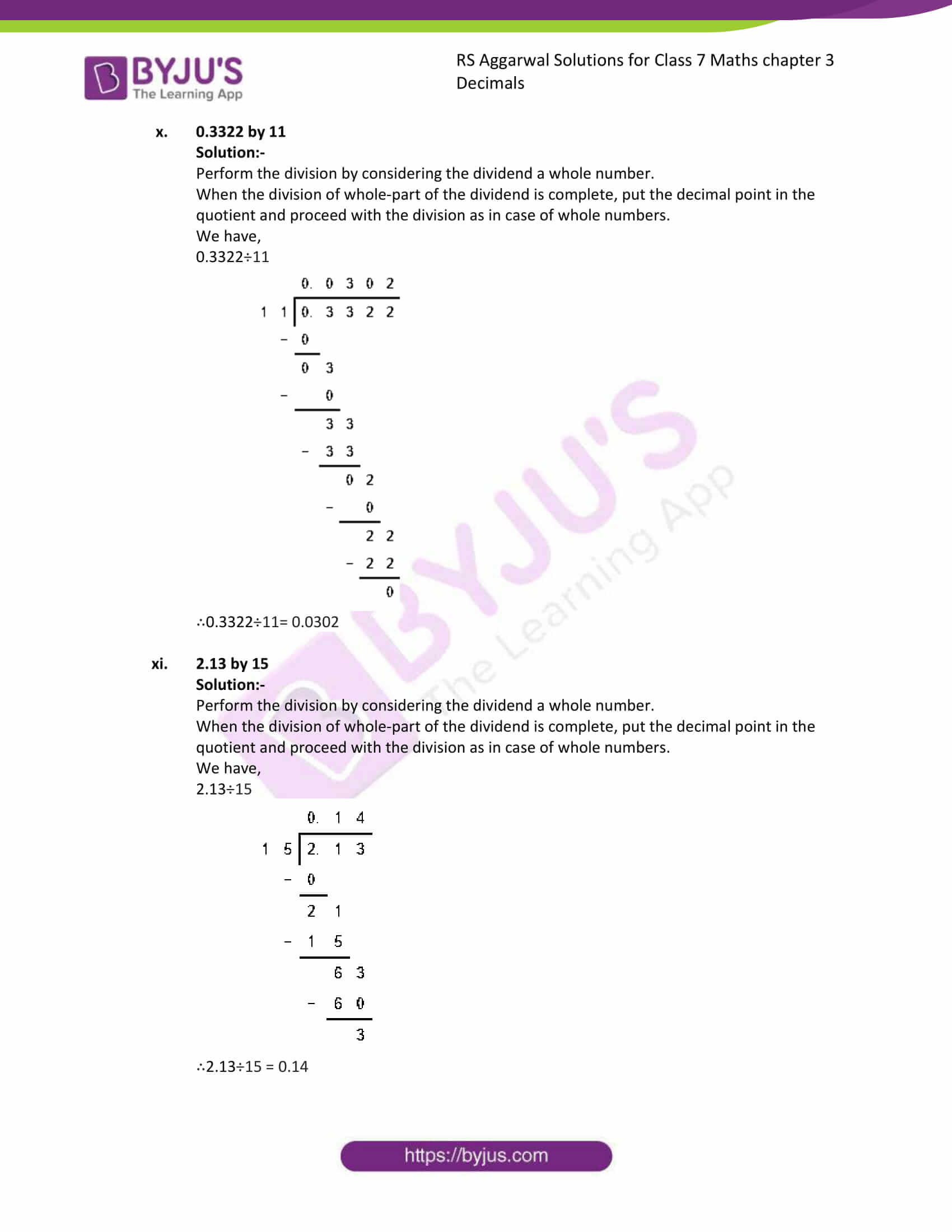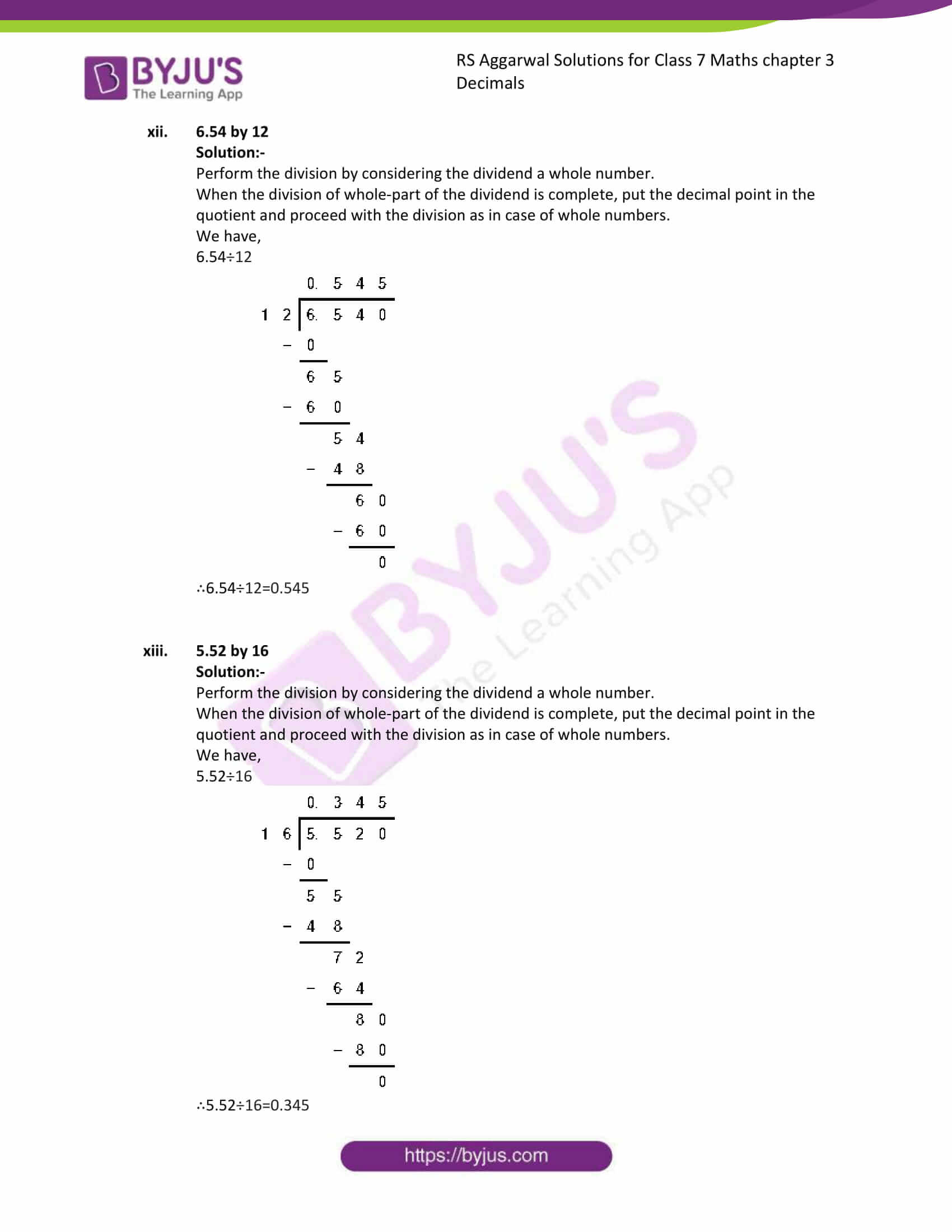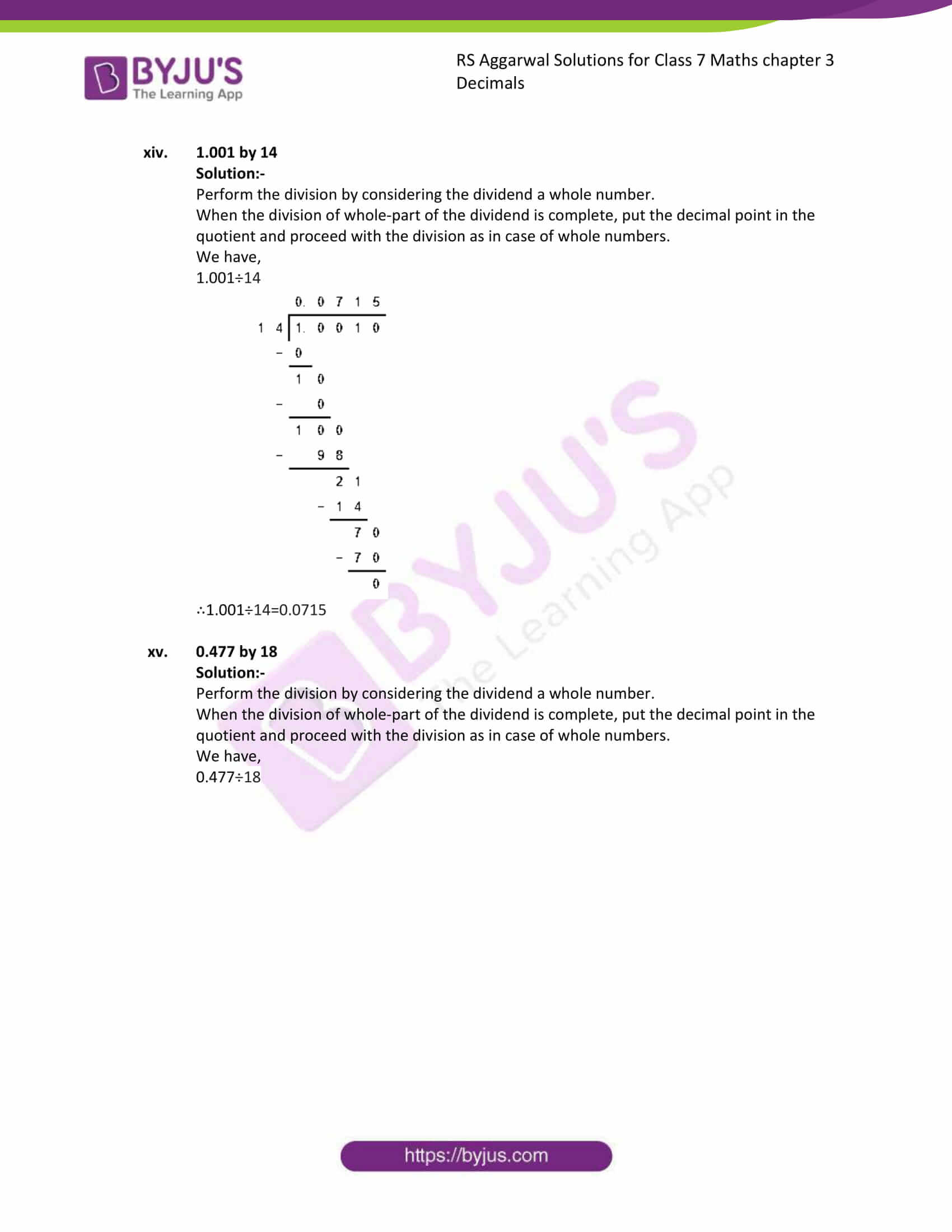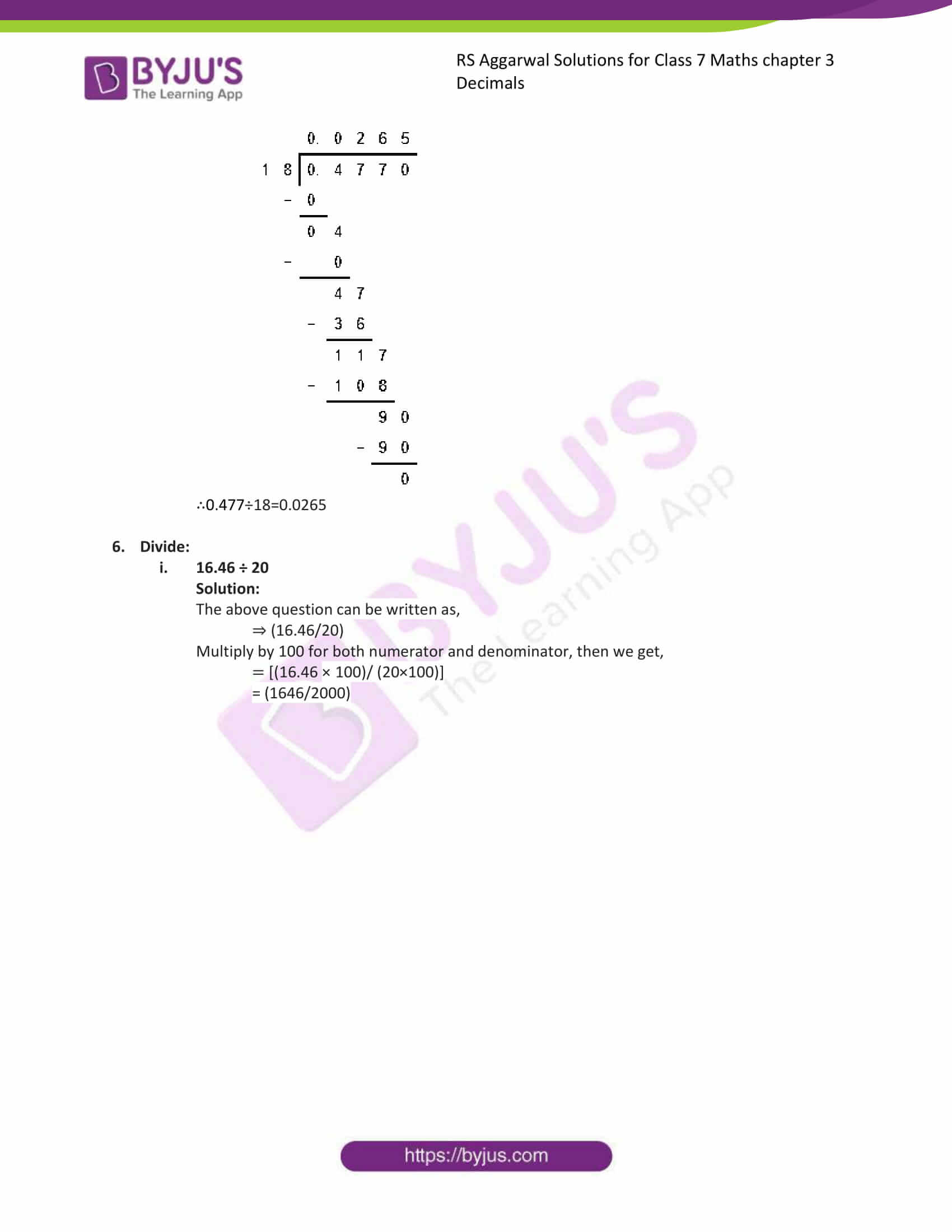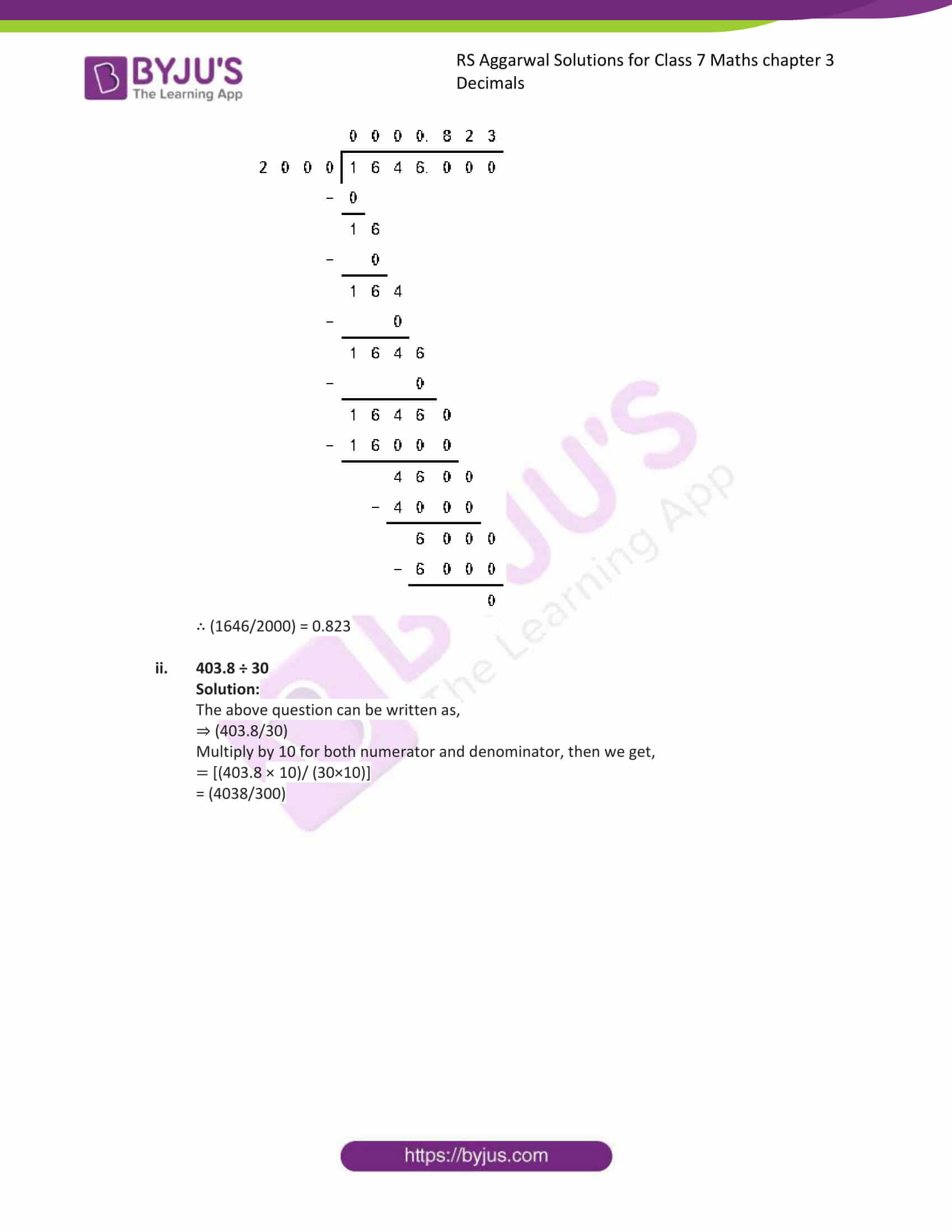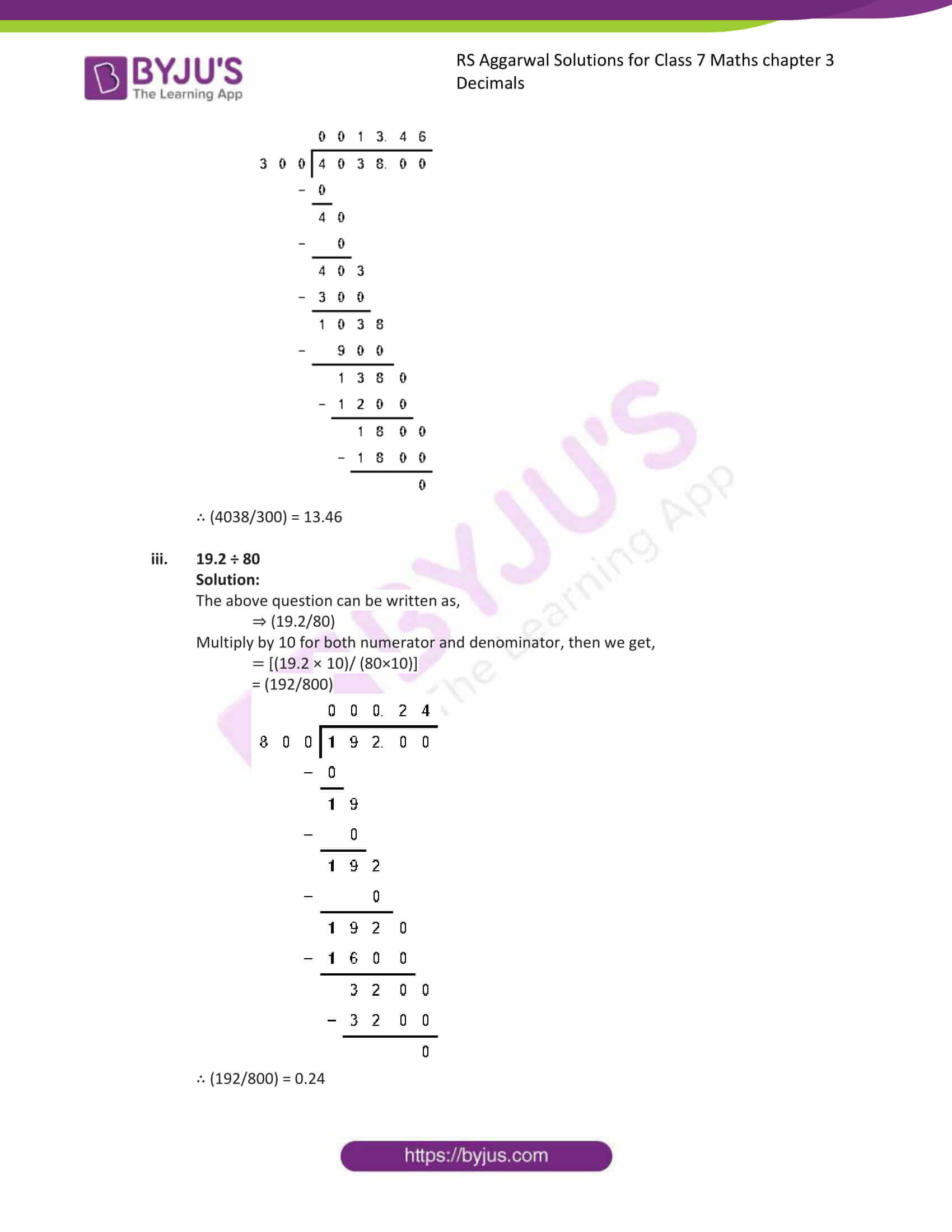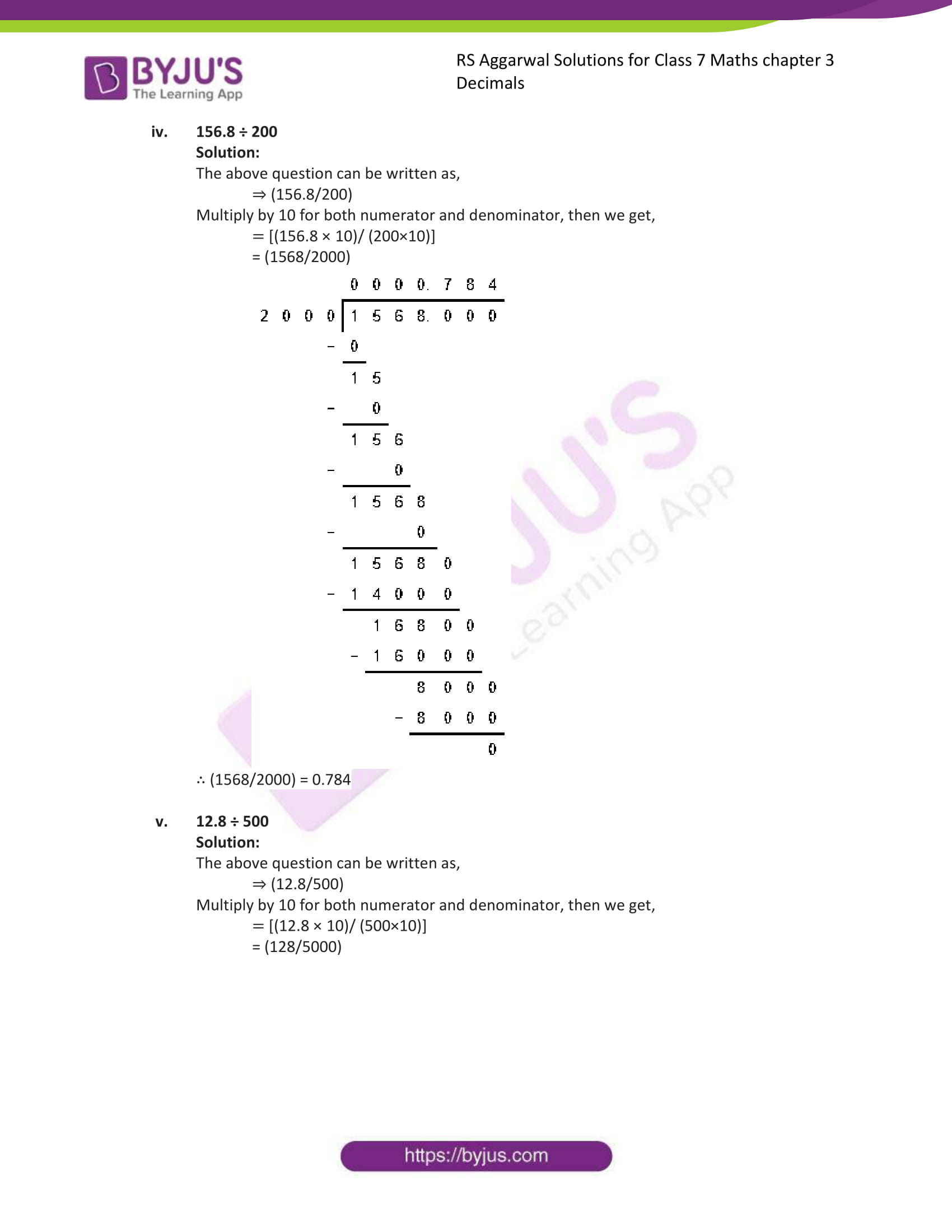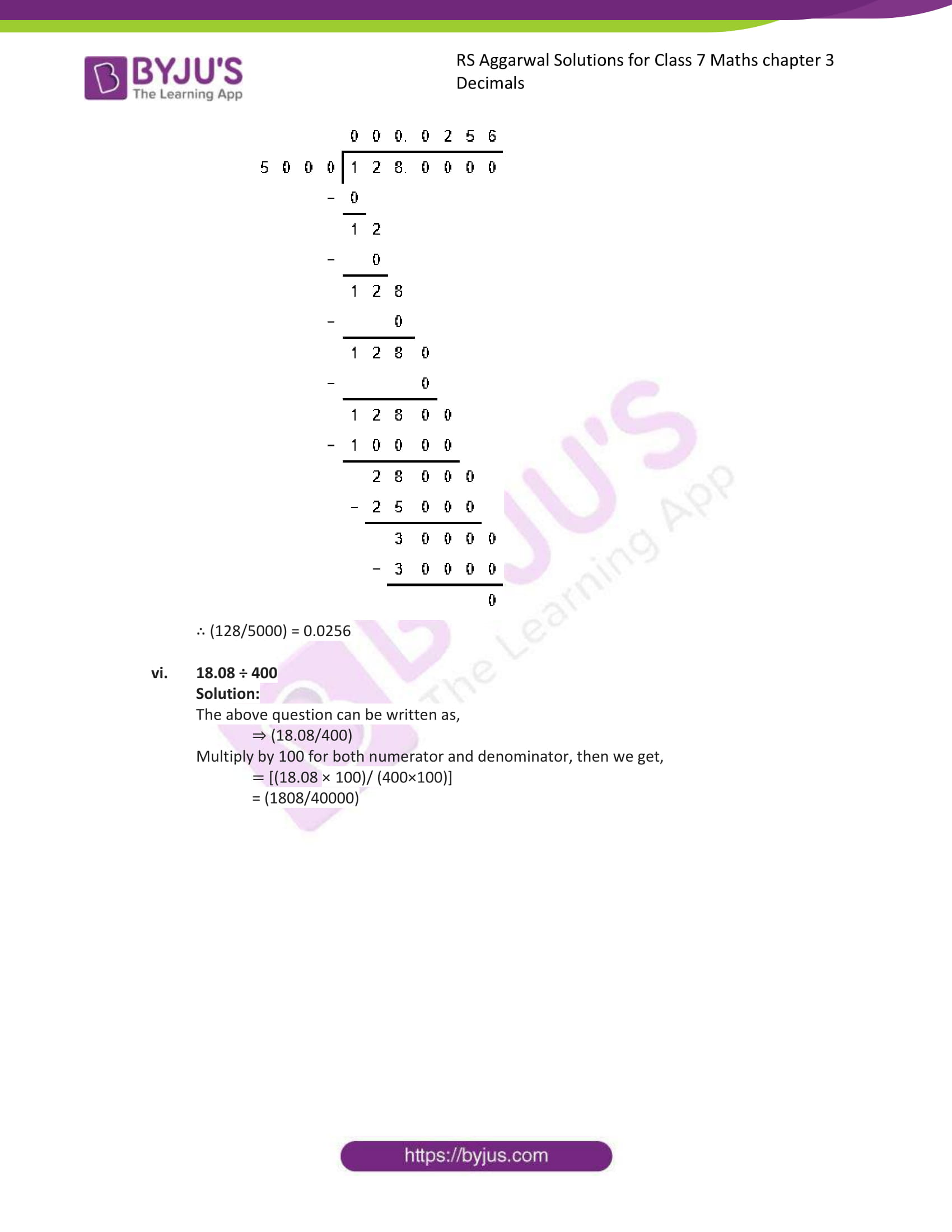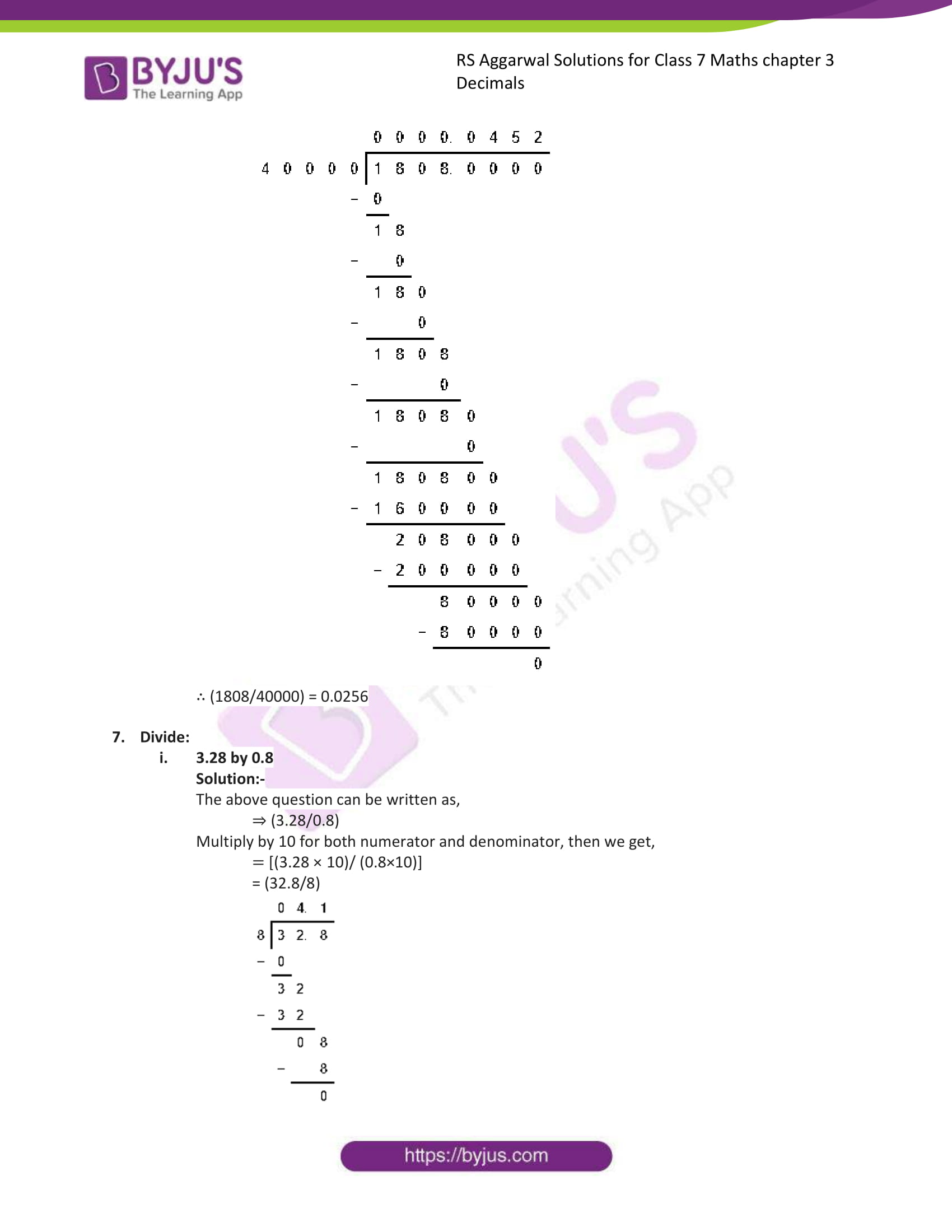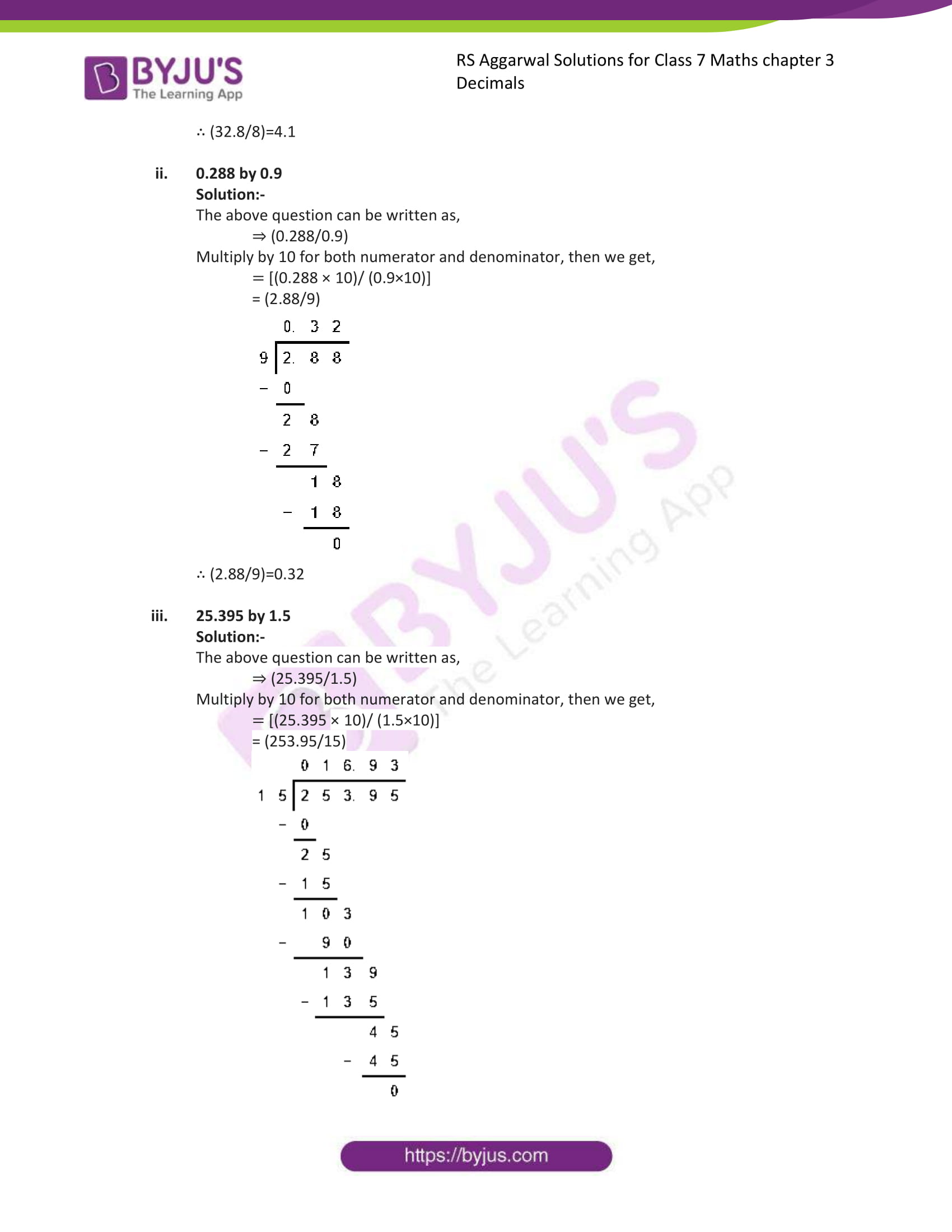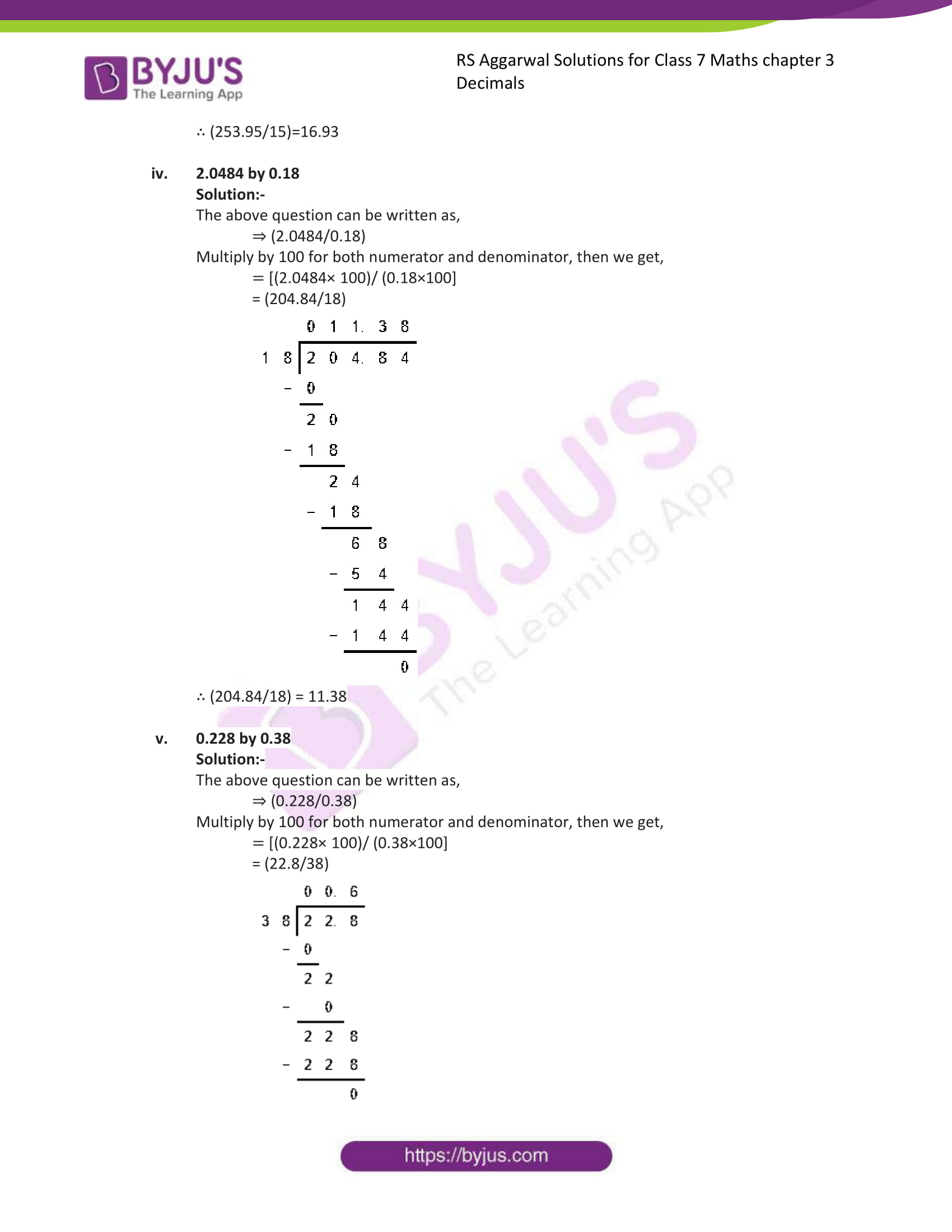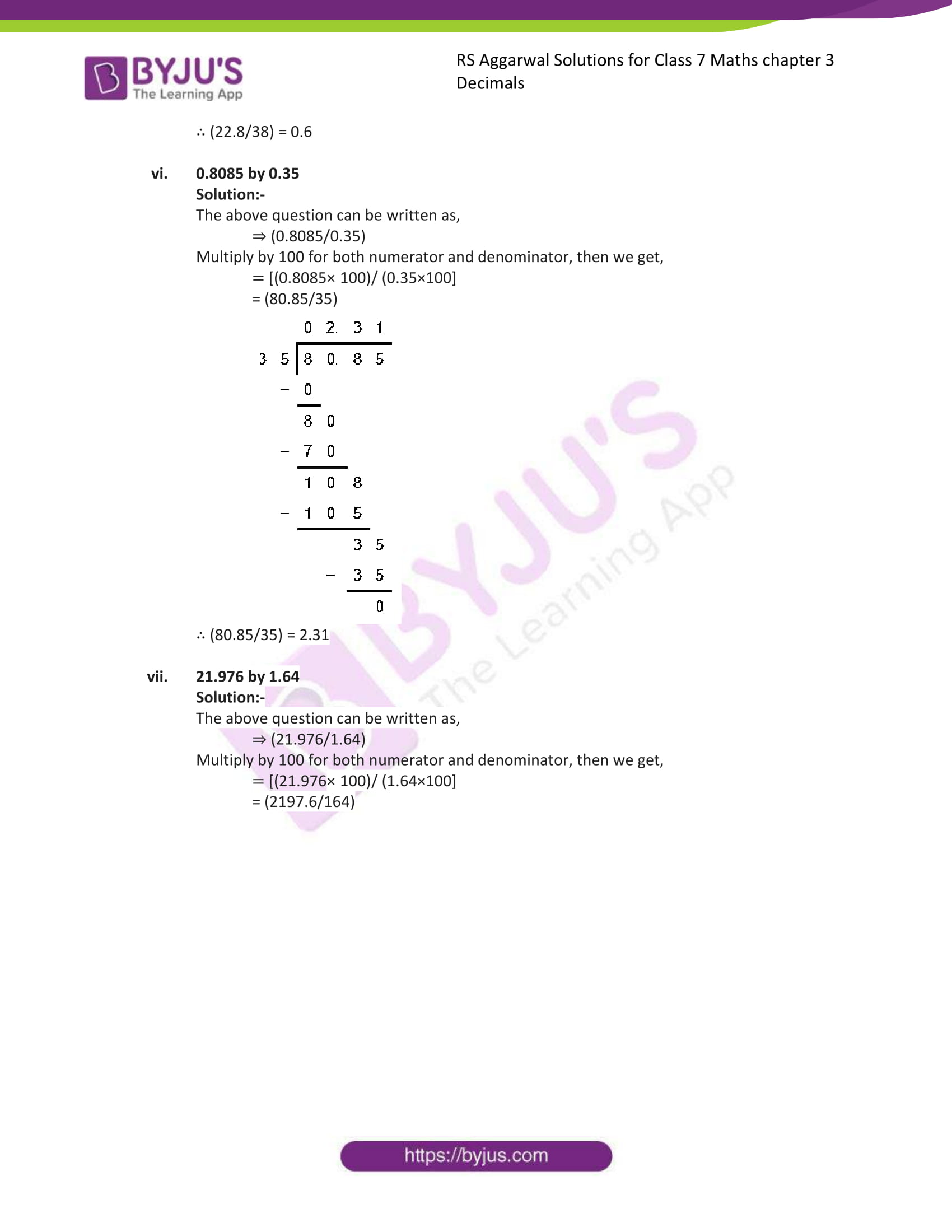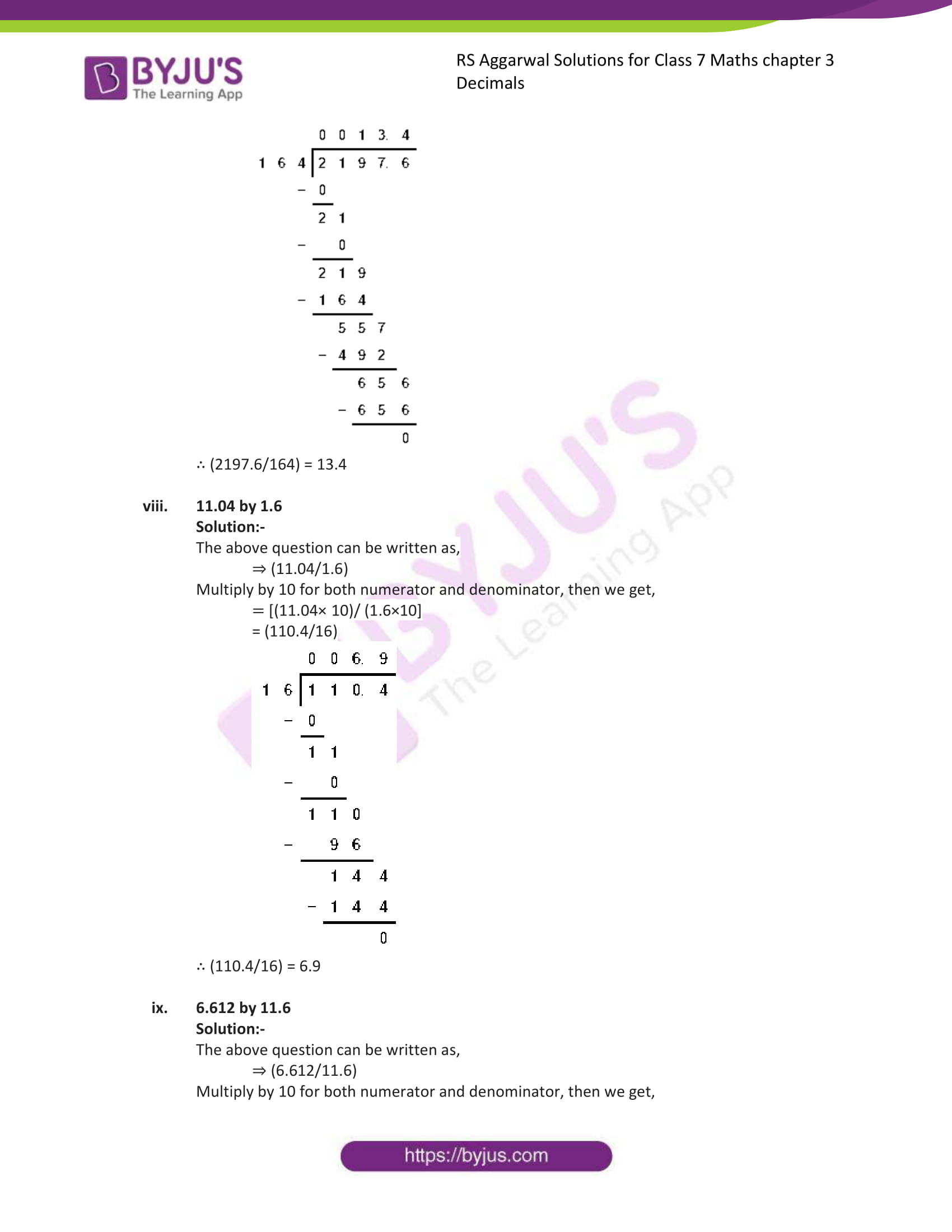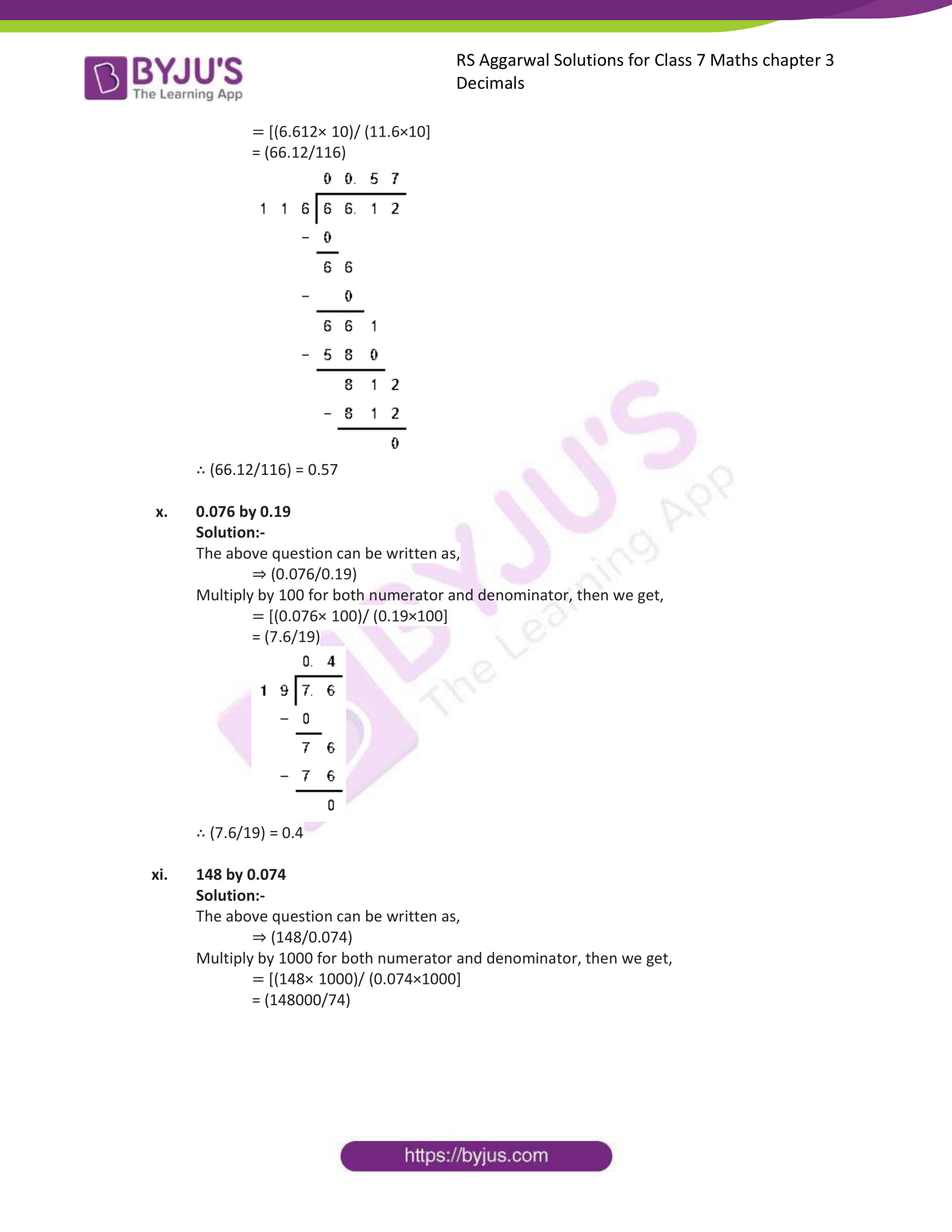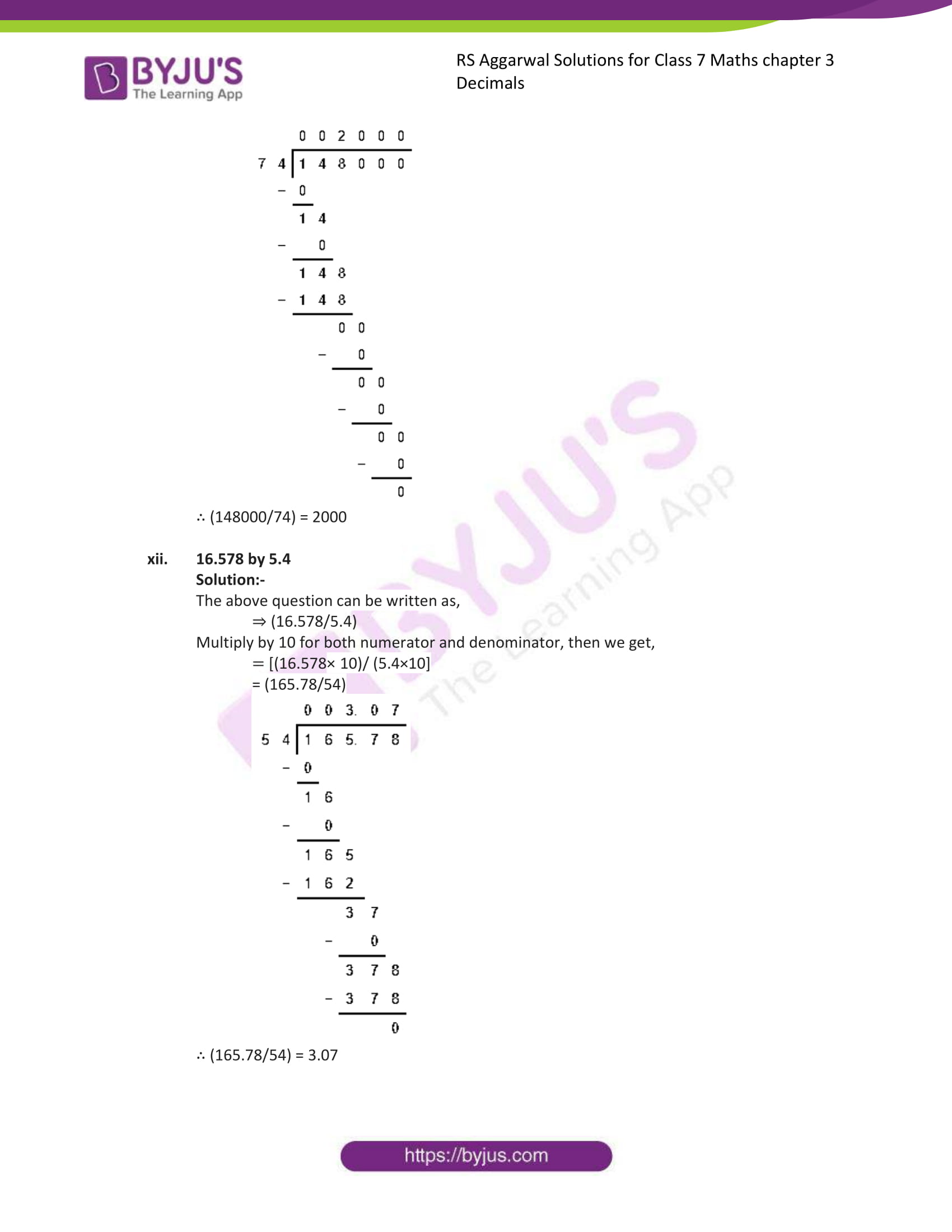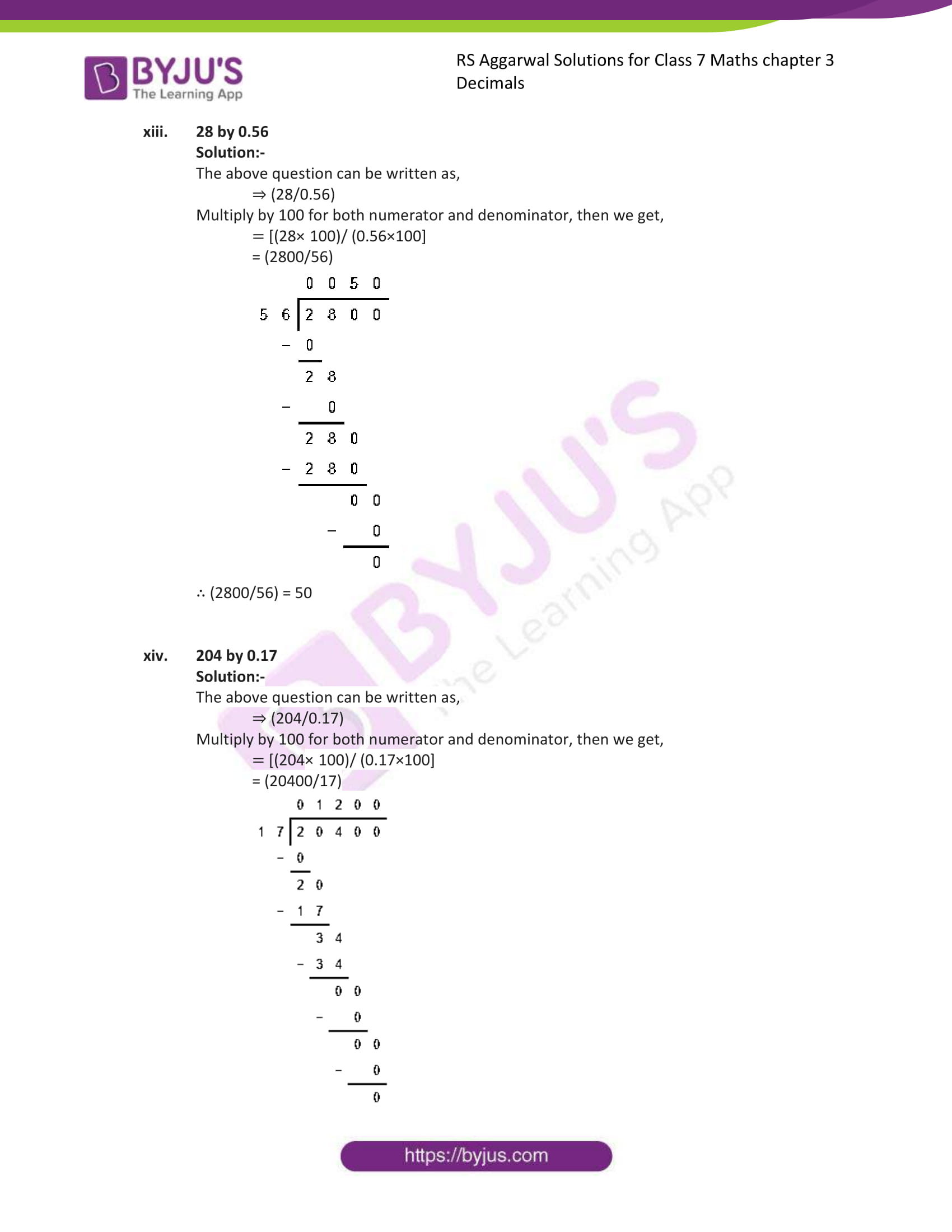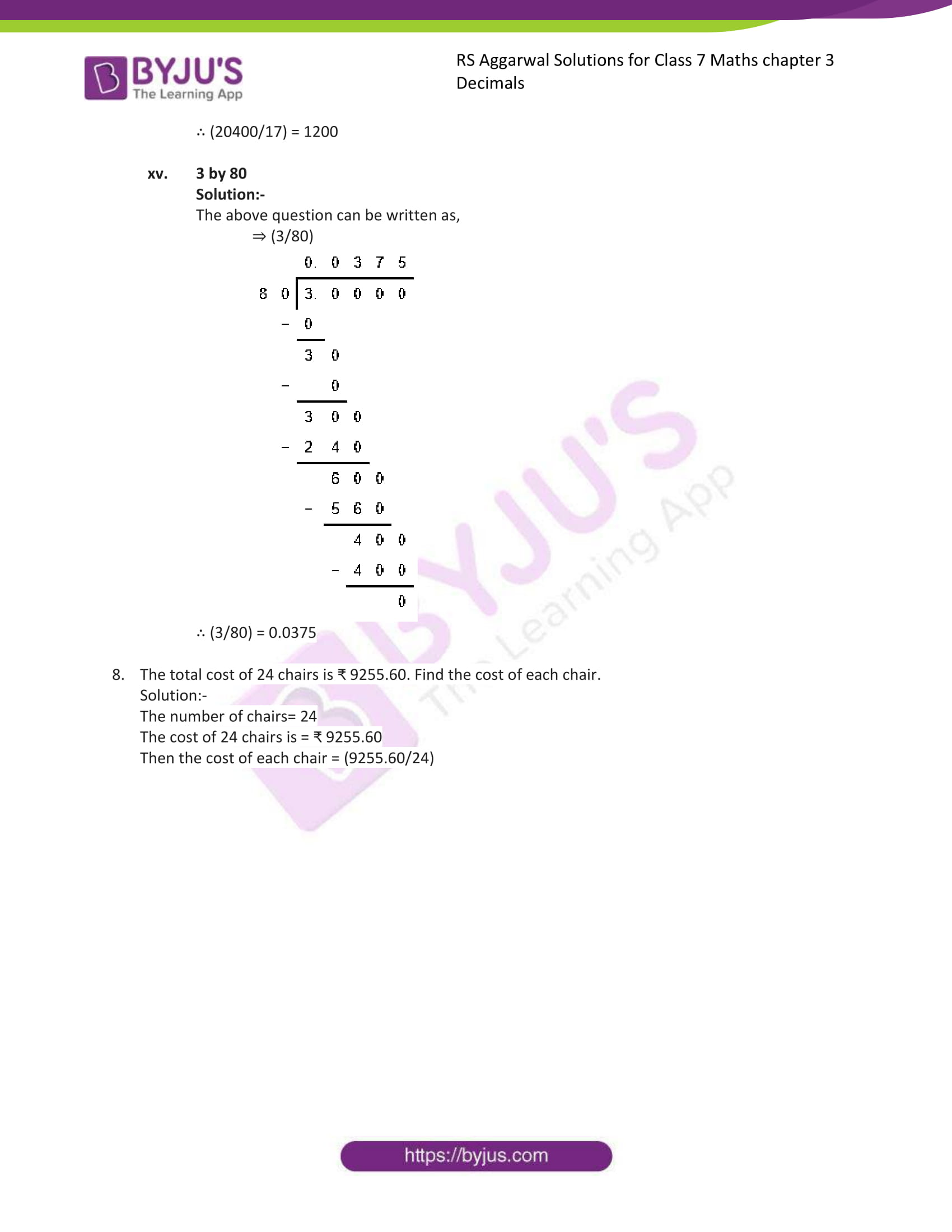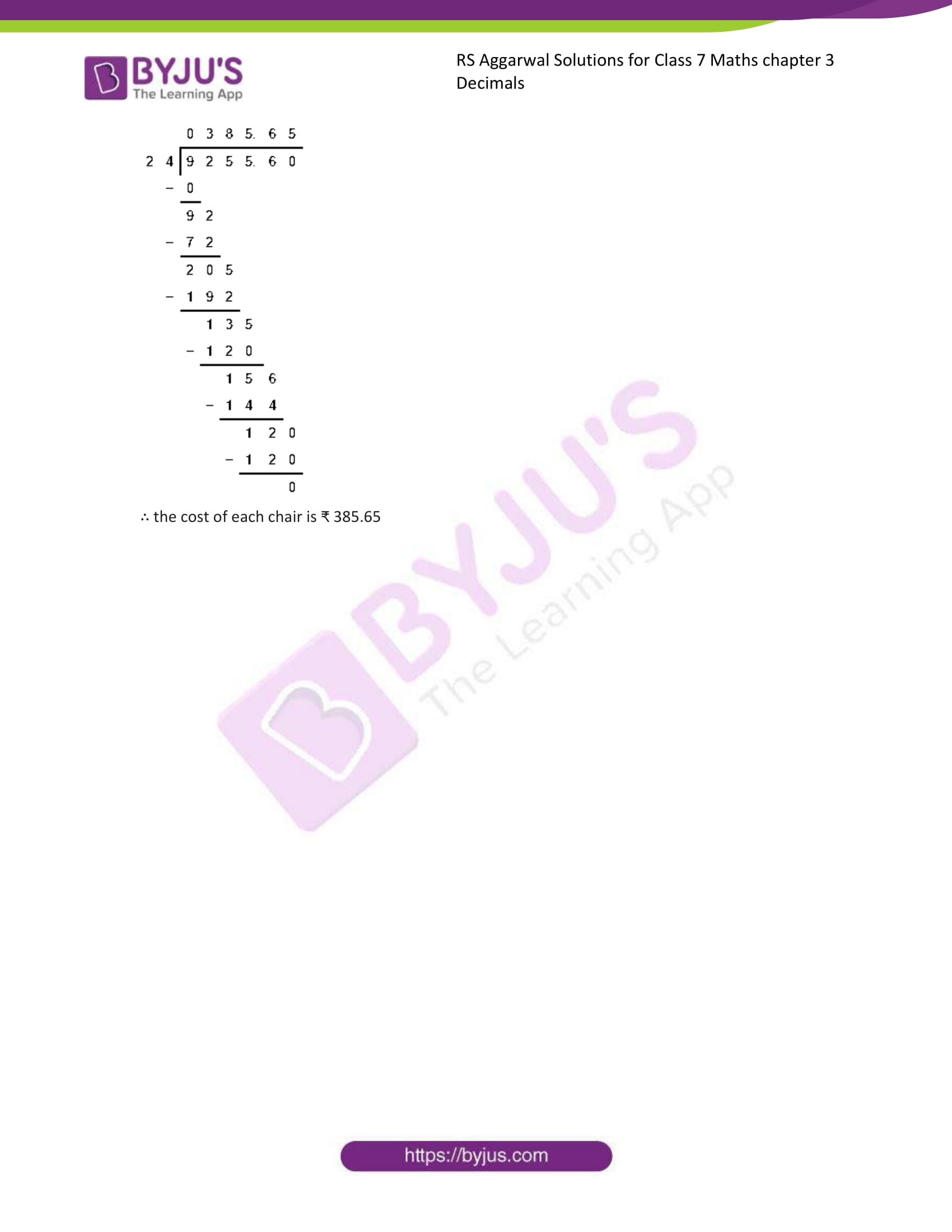### Access answers to Maths RS Aggarwal Solutions for Class 7 Chapter 3 – Decimals Exercise 3D

1. Divide:

(i) 131.6 by 10

Solution:-

On dividing a decimal by 10, the decimal point is shifted to the left by one place.

We have,

= 131.6 ÷ 10

= (131.6/10)

= 13.16

(ii) 32.56 by 10

Solution:-

On dividing a decimal by 10, the decimal point is shifted to the left by one place.

We have,

= 32.56 ÷ 10

= (32.56/10)

= 3.256

(iii) 4.38 by 10

Solution:-

On dividing a decimal by 10, the decimal point is shifted to the left by one place.

We have,

= 4.38 ÷ 10

= (4.38/10)

= 0.438

(iv) 0.34 by 10

Solution:-

On dividing a decimal by 10, the decimal point is shifted to the left by one place.

We have,

= 0.34 ÷ 10

= (0.34/10)

= 0.034

(v) 0.08 by 10

Solution:-

On dividing a decimal by 10, the decimal point is shifted to the left by one place.

We have,

= 0.08 ÷ 10

= (0.08/10)

= 0.008

(vi) 0.062 by 10

Solution:-

On dividing a decimal by 10, the decimal point is shifted to the left by one place.

We have,

= 0.062 ÷ 10

= (0.062/10)

= 0.0062

2. Divide:

(i) 137.2 by 100

Solution:-

On dividing a decimal by 100, the decimal point is shifted to the left by two places.

We have,

= 137.2 ÷ 100

= (137.2/100)

= 1.372

(ii) 23.4 by 100

Solution:-

On dividing a decimal by 100, the decimal point is shifted to the left by two places.

We have,

= 23.4 ÷ 100

= (23.4/100)

= 0.234

(iii) 4.7 by 100

Solution:-

On dividing a decimal by 100, the decimal point is shifted to the left by two places.

We have,

= 4.7 ÷ 100

= (4.7/100)

= 0.047

(iv) 0.3 by 100

Solution:-

On dividing a decimal by 100, the decimal point is shifted to the left by two places.

We have,

= 0.3 ÷ 100

= (0.3/100)

= 0.003

(v) 0.58 by 100

Solution:-

On dividing a decimal by 100, the decimal point is shifted to the left by two places.

We have,

= 0.58 ÷ 100

= (0.58/100)

= 0.0058

(vi) 0.02 by 100

Solution:-

On dividing a decimal by 100, the decimal point is shifted to the left by two places.

We have,

= 0.02 ÷ 100

= (0.02/100)

= 0.0002

3. Divide:

(i) 1286.5 by 1000

Solution:-

On dividing a decimal by 1000, the decimal point is shifted to the left by three places.

We have,

= 1286.5 ÷ 1000

= (1286.5/1000)

= 1.2865

(ii) 354.16 by 1000

Solution:-

On dividing a decimal by 1000, the decimal point is shifted to the left by three places.

We have,

= 354.16 ÷ 1000

= (354.16/1000)

= 0.35416

(iii) 38.9 by 1000

Solution:-

On dividing a decimal by 1000, the decimal point is shifted to the left by three places.

We have,

= 38.9 ÷ 1000

= (38.9/1000)

= 0.0389

(iv) 4.6 by 1000

Solution:-

On dividing a decimal by 1000, the decimal point is shifted to the left by three places.

We have,

= 4.6 ÷ 1000

= (4.6/1000)

= 0.0046

(v) 0.8 by 1000

Solution:-

On dividing a decimal by 1000, the decimal point is shifted to the left by three places.

We have,

= 0.8 ÷ 1000

= (0.8/1000)

= 0.0008

(vi) 2 by 1000

Solution:-

On dividing a decimal by 1000, the decimal point is shifted to the left by three places.

We have,

= 2 ÷ 1000

= (2/1000)

= 0.0002

4. Divide:-

(i) 12 by 8

Solution:-

The above question can be written as, 12 ÷ 8

Then,

= (12/8) … [÷2]

= (3/2)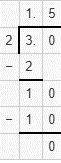∴12 ÷ 8 = 1.5

(ii) 63 by 15

Solution:-

The above question can be written as, 63 ÷ 15

Then,

= (63/15) … [÷3]

= (21/5)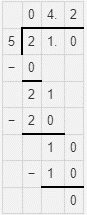∴63 ÷ 15 = 1.5

(iii) 47 by 20

Solution:-

The above question can be written as, 47 ÷ 20

Then,

= (47/20)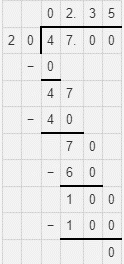∴47 ÷ 20 = 2.35

(iv) 101 by 25

Solution:-

The above question can be written as, 101 ÷ 25

Then,

= (101/25)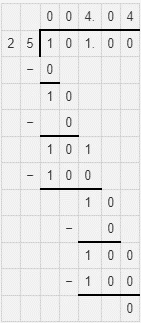∴101 ÷ 25 = 4.04

(v) 31 by 40

Solution:-

The above question can be written as, 31 ÷ 40

Then,

= (31/40)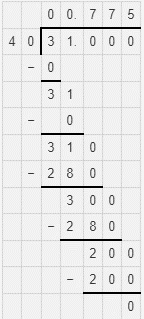∴31 ÷ 40= 0.775

(vi) 11 by 16

Solution:-

The above question can be written as, 11 ÷ 16

Then,

= (31/40)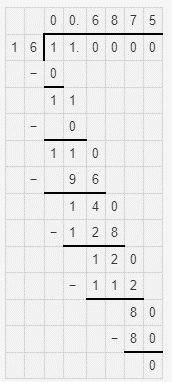∴11 ÷ 16=0.06875

5. Divide:

(i) 43.2 by 6

Solution:-

Perform the division by considering the dividend a whole number.

When the division of whole-part of the dividend is complete, put the decimal point in the quotient and proceed with the division as in case of whole numbers.

We have,

43.2÷6∴43.2÷6 = 7.2

(ii) 60.48 by 12

Solution:-

Perform the division by considering the dividend a whole number.

When the division of whole-part of the dividend is complete, put the decimal point in the quotient and proceed with the division as in case of whole numbers.

We have,

60.48÷12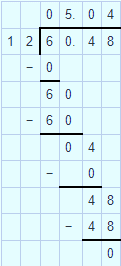∴60.48÷12 = 5.04

(iii) 117.6 by 21

Solution:-

Perform the division by considering the dividend a whole number.

When the division of whole-part of the dividend is complete, put the decimal point in the quotient and proceed with the division as in case of whole numbers.

We have,

117.6÷21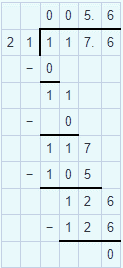∴117.6÷21=5.6

(iv) 217.44 by 18

Solution:-

Perform the division by considering the dividend a whole number.

When the division of whole-part of the dividend is complete, put the decimal point in the quotient and proceed with the division as in case of whole numbers.

We have,

217.44÷18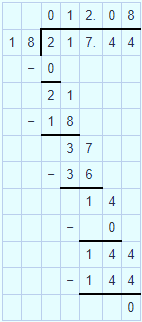∴217.44÷18=12.08

(v) 2.575 by 25

Solution:-

Perform the division by considering the dividend a whole number.

When the division of whole-part of the dividend is complete, put the decimal point in the quotient and proceed with the division as in case of whole numbers.

We have,

2.575÷25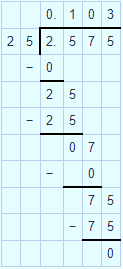∴2.575÷25= 0.103

(vi) 6.08 by 8

Solution:-

Perform the division by considering the dividend a whole number.

When the division of whole-part of the dividend is complete, put the decimal point in the quotient and proceed with the division as in case of whole numbers.

We have,

6.08÷6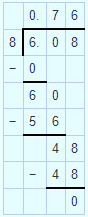∴6.08÷6=0.76

(vii) 0.765 by 9

Solution:-

Perform the division by considering the dividend a whole number.

When the division of whole-part of the dividend is complete, put the decimal point in the quotient and proceed with the division as in case of whole numbers.

We have,

0.765÷9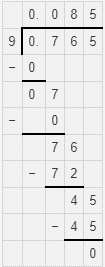∴0.765÷9=0.085

(viii) 0.768 by16

Solution:-

Perform the division by considering the dividend a whole number.

When the division of whole-part of the dividend is complete, put the decimal point in the quotient and proceed with the division as in case of whole numbers.

We have,

0.768÷16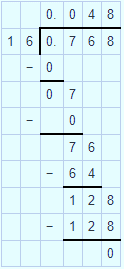∴0.769÷16=0.048

(ix) 0.175 by 25

Solution:-

Perform the division by considering the dividend a whole number.

When the division of whole-part of the dividend is complete, put the decimal point in the quotient and proceed with the division as in case of whole numbers.

We have,

0.175÷25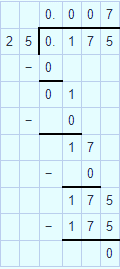∴0.175÷25= 0.007

(x) 0.3322 by 11

Solution:-

Perform the division by considering the dividend a whole number.

When the division of whole-part of the dividend is complete, put the decimal point in the quotient and proceed with the division as in case of whole numbers.

We have,

0.3322÷11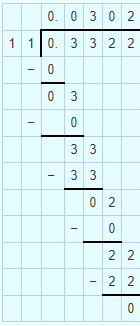∴0.3322÷11= 0.0302

(xi) 2.13 by 15

Solution:-

Perform the division by considering the dividend a whole number.

When the division of whole-part of the dividend is complete, put the decimal point in the quotient and proceed with the division as in case of whole numbers.

We have,

2.13÷15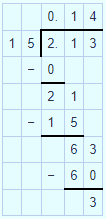∴2.13÷15 = 0.14

(xii) 6.54 by 12

Solution:-

Perform the division by considering the dividend a whole number.

When the division of whole-part of the dividend is complete, put the decimal point in the quotient and proceed with the division as in case of whole numbers.

We have,

6.54÷12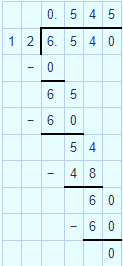∴6.54÷12=0.545

(xiii) 5.52 by 16

Solution:-

Perform the division by considering the dividend a whole number.

When the division of whole-part of the dividend is complete, put the decimal point in the quotient and proceed with the division as in case of whole numbers.

We have,

5.52÷16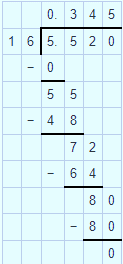∴5.52÷16=0.345

(xiv) 1.001 by 14

Solution:-

Perform the division by considering the dividend a whole number.

When the division of whole-part of the dividend is complete, put the decimal point in the quotient and proceed with the division as in case of whole numbers.

We have,

1.001÷14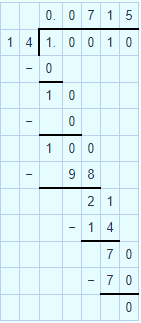∴1.001÷14=0.0715

(xv) 0.477 by 18

Solution:-

Perform the division by considering the dividend a whole number.

When the division of whole-part of the dividend is complete, put the decimal point in the quotient and proceed with the division as in case of whole numbers.

We have,

0.477÷18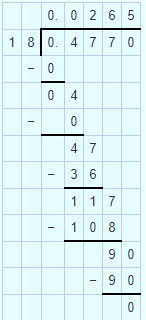∴0.477÷18=0.0265

6. Divide:

(i) 16.46 ÷ 20

Solution:

The above question can be written as,

⇒ (16.46/20)

Multiply by 100 for both numerator and denominator, then we get,

= [(16.46 × 100)/ (20×100)]

= (1646/2000)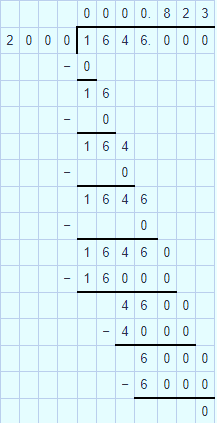∴ (1646/2000) = 0.823

(ii) 403.8 ÷ 30

Solution:

The above question can be written as,

⇒ (403.8/30)

Multiply by 10 for both numerator and denominator, then we get,

= [(403.8 × 10)/ (30×10)]

= (4038/300)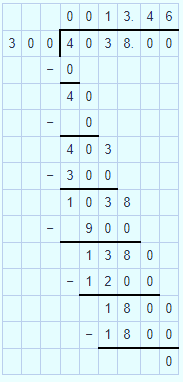∴ (4038/300) = 13.46

(iii) 19.2 ÷ 80

Solution:

The above question can be written as,

⇒ (19.2/80)

Multiply by 10 for both numerator and denominator, then we get,

= [(19.2 × 10)/ (80×10)]

= (192/800)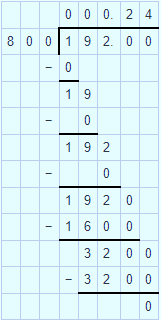∴ (192/800) = 0.24

(iv) 156.8 ÷ 200

Solution:

The above question can be written as,

⇒ (156.8/200)

Multiply by 10 for both numerator and denominator, then we get,

= [(156.8 × 10)/ (200×10)]

= (1568/2000)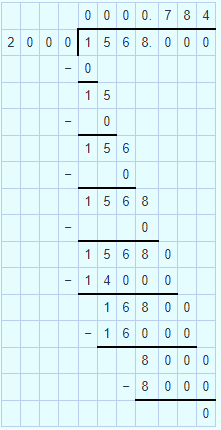∴ (1568/2000) = 0.784

(v) 12.8 ÷ 500

Solution:

The above question can be written as,

⇒ (12.8/500)

Multiply by 10 for both numerator and denominator, then we get,

= [(12.8 × 10)/ (500×10)]

= (128/5000)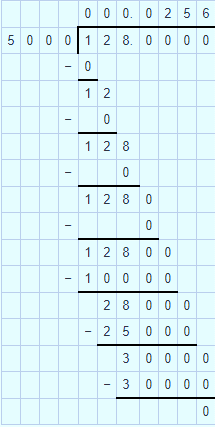∴ (128/5000) = 0.0256

(vi) 18.08 ÷ 400

Solution:

The above question can be written as,

⇒ (18.08/400)

Multiply by 100 for both numerator and denominator, then we get,

= [(18.08 × 100)/ (400×100)]

= (1808/40000)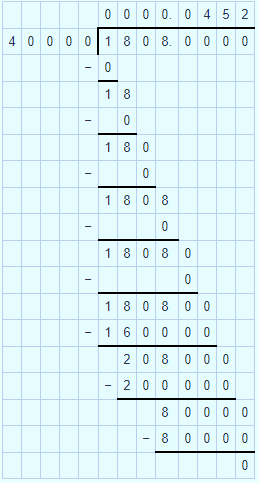∴ (1808/40000) = 0.0256

7. Divide:

(i) 3.28 by 0.8

Solution:-

The above question can be written as,

⇒ (3.28/0.8)

Multiply by 10 for both numerator and denominator, then we get,

= [(3.28 × 10)/ (0.8×10)]

= (32.8/8)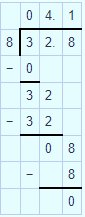∴ (32.8/8)=4.1

(ii) 0.288 by 0.9

Solution:-

The above question can be written as,

⇒ (0.288/0.9)

Multiply by 10 for both numerator and denominator, then we get,

= [(0.288 × 10)/ (0.9×10)]

= (2.88/9)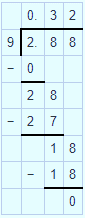∴ (2.88/9)=0.32

(iii) 25.395 by 1.5

Solution:-

The above question can be written as,

⇒ (25.395/1.5)

Multiply by 10 for both numerator and denominator, then we get,

= [(25.395 × 10)/ (1.5×10)]

= (253.95/15)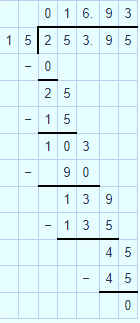∴ (253.95/15)=16.93

(iv) 2.0484 by 0.18

Solution:-

The above question can be written as,

⇒ (2.0484/0.18)

Multiply by 100 for both numerator and denominator, then we get,

= [(2.0484× 100)/ (0.18×100]

= (204.84/18)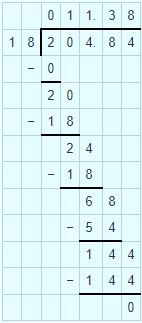∴ (204.84/18) = 11.38

(v) 0.228 by 0.38

Solution:-

The above question can be written as,

⇒ (0.228/0.38)

Multiply by 100 for both numerator and denominator, then we get,

= [(0.228× 100)/ (0.38×100]

= (22.8/38)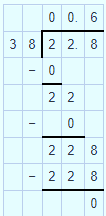∴ (22.8/38) = 0.6

(vi) 0.8085 by 0.35

Solution:-

The above question can be written as,

⇒ (0.8085/0.35)

Multiply by 100 for both numerator and denominator, then we get,

= [(0.8085× 100)/ (0.35×100]

= (80.85/35)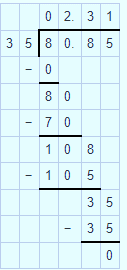∴ (80.85/35) = 2.31

(vii) 21.976 by 1.64

Solution:-

The above question can be written as,

⇒ (21.976/1.64)

Multiply by 100 for both numerator and denominator, then we get,

= [(21.976× 100)/ (1.64×100]

= (2197.6/164)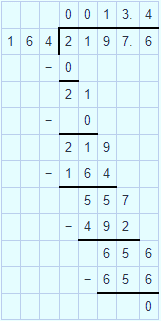∴ (2197.6/164) = 13.4

(viii) 11.04 by 1.6

Solution:-

The above question can be written as,

⇒ (11.04/1.6)

Multiply by 10 for both numerator and denominator, then we get,

= [(11.04× 10)/ (1.6×10]

= (110.4/16)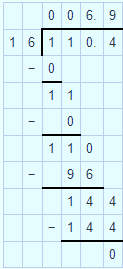∴ (110.4/16) = 6.9

(ix) 6.612 by 11.6

Solution:-

The above question can be written as,

⇒ (6.612/11.6)

Multiply by 10 for both numerator and denominator, then we get,

= [(6.612× 10)/ (11.6×10]

= (66.12/116)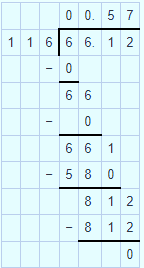∴ (66.12/116) = 0.57

(x) 0.076 by 0.19

Solution:-

The above question can be written as,

⇒ (0.076/0.19)

Multiply by 100 for both numerator and denominator, then we get,

= [(0.076× 100)/ (0.19×100]

= (7.6/19)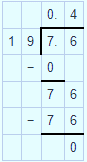∴ (7.6/19) = 0.4

(xi) 148 by 0.074

Solution:-

The above question can be written as,

⇒ (148/0.074)

Multiply by 1000 for both numerator and denominator, then we get,

= [(148× 1000)/ (0.074×1000]

= (148000/74)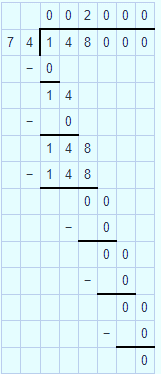∴ (148000/74) = 2000

(xii) 16.578 by 5.4

Solution:-

The above question can be written as,

⇒ (16.578/5.4)

Multiply by 10 for both numerator and denominator, then we get,

= [(16.578× 10)/ (5.4×10]

= (165.78/54)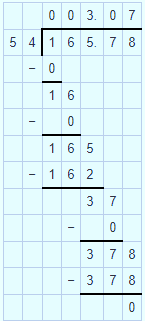∴ (165.78/54) = 3.07

(xiii) 28 by 0.56

Solution:-

The above question can be written as,

⇒ (28/0.56)

Multiply by 100 for both numerator and denominator, then we get,

= [(28× 100)/ (0.56×100]

= (2800/56)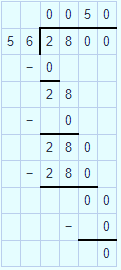∴ (2800/56) = 50

(xiv) 204 by 0.17

Solution:-

The above question can be written as,

⇒ (204/0.17)

Multiply by 100 for both numerator and denominator, then we get,

= [(204× 100)/ (0.17×100]

= (20400/17)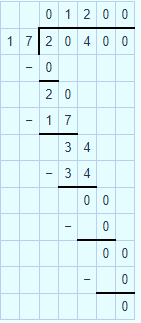∴ (20400/17) = 1200

(xv) 3 by 80

Solution:-

The above question can be written as,

⇒ (3/80)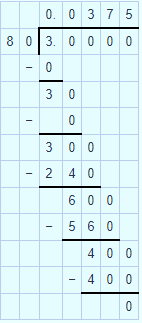∴ (3/80) = 0.0375

8. The total cost of 24 chairs is ₹ 9255.60. Find the cost of each chair.

Solution:-

The number of chairs= 24

The cost of 24 chairs is = ₹ 9255.60

Then the cost of each chair = (9255.60/24)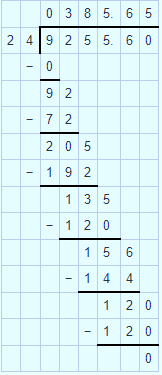∴ the cost of each chair is ₹ 385.65

### Access other exercises of RS Aggarwal Solutions For Class 7 Chapter 3 – Decimals

Exercise 3E Solutions